# Electro-magnetism

## Exercise 10(A)

#### Question 1

By using a compass needle describe how can you demonstrate that there is a magnetic field around a current carrying conductor.

Experiment:

In figure below, AB is a wire lying in the north-south direction and connected to a battery through a rheostat and a tapping key. A compass needle is placed just below the wire.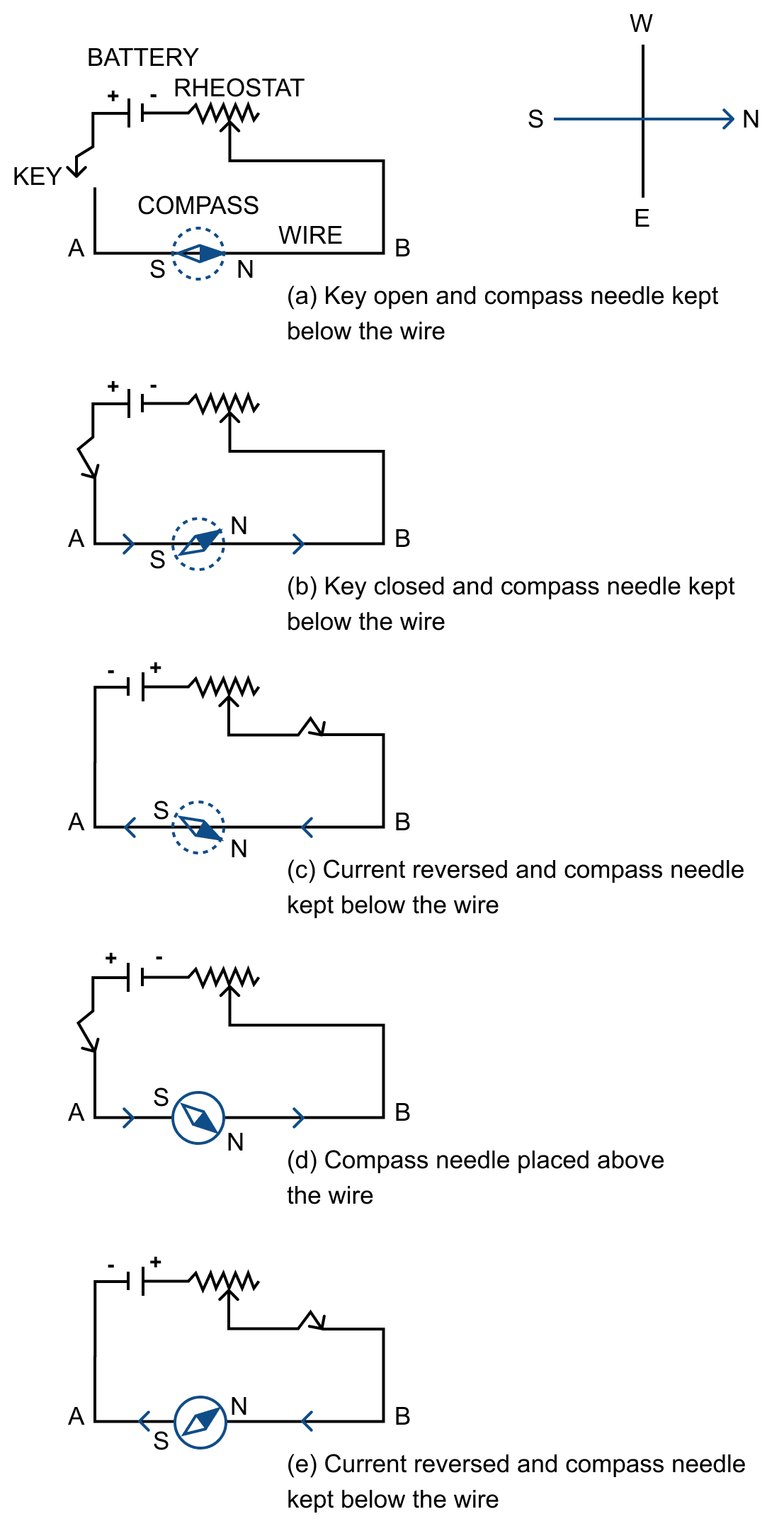Observation —

(1) When the key is open i.e., no current passes through the wire, the needle points in the N-S direction (i.e., along the earth's magnetic field) with the north pole of needle pointing towards the north direction. In this position, the needle is parallel to the wire as shown in Fig. (a).

(2) When the key is pressed, a current passes in the wire in the direction from A to B (i.e. from south to north) and the north pole (N) of the needle deflects towards the west [Fig.(b)].

(3) When the direction of current in the wire is reversed by reversing the connections at the terminals of the battery, north pole (N) of the needle deflects towards the east [Fig.(c)].

(4) If the compass needle is placed just above the wire, the north pole (N) deflects towards east when the direction of current in wire is from A to B [Fig. (d)], but the needle deflects towards west as in figure (e), if the direction of current in wire is from B to A.

Through the experiment we can say that, when electric current is passed through a conducting wire, a magnetic field is produced around it.

#### Question 2

Draw a diagram showing the direction of three magnetic field lines due to a straight wire carrying current. Also show the direction of current in the wire.

Below diagram shows the direction of three magnetic field lines due to a straight wire carrying current: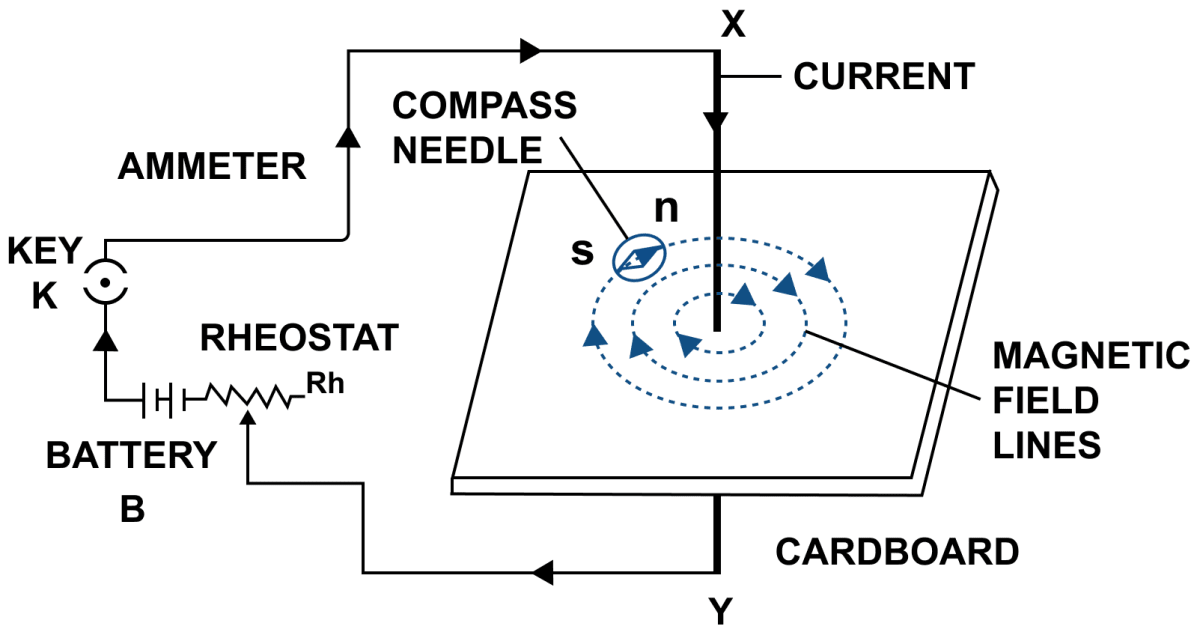The current in the wire is in clockwise direction.

#### Question 3

How is magnetic field due to a straight current carrying wire affected if current in wire is (a) decreased, (b) reversed?

(a) On decreasing current in the wire, the deflection of compass needle decreases which implies that the strength of magnetic field around the wire decreases.

(b) On reversing the direction of current the magnetic needle of compass reverses because the direction of magnetic field reverses.

#### Question 4

State a law, which determines the direction of magnetic field around a current carrying wire.

Right hand thumb rule is used to determine the direction of magnetic field around a current carrying wire.

Right hand thumb rule — If we hold the current carrying conductor in our right hand such that the thumb points in the direction of flow of current, then the fingers encircle the wire in the direction of the magnetic field lines.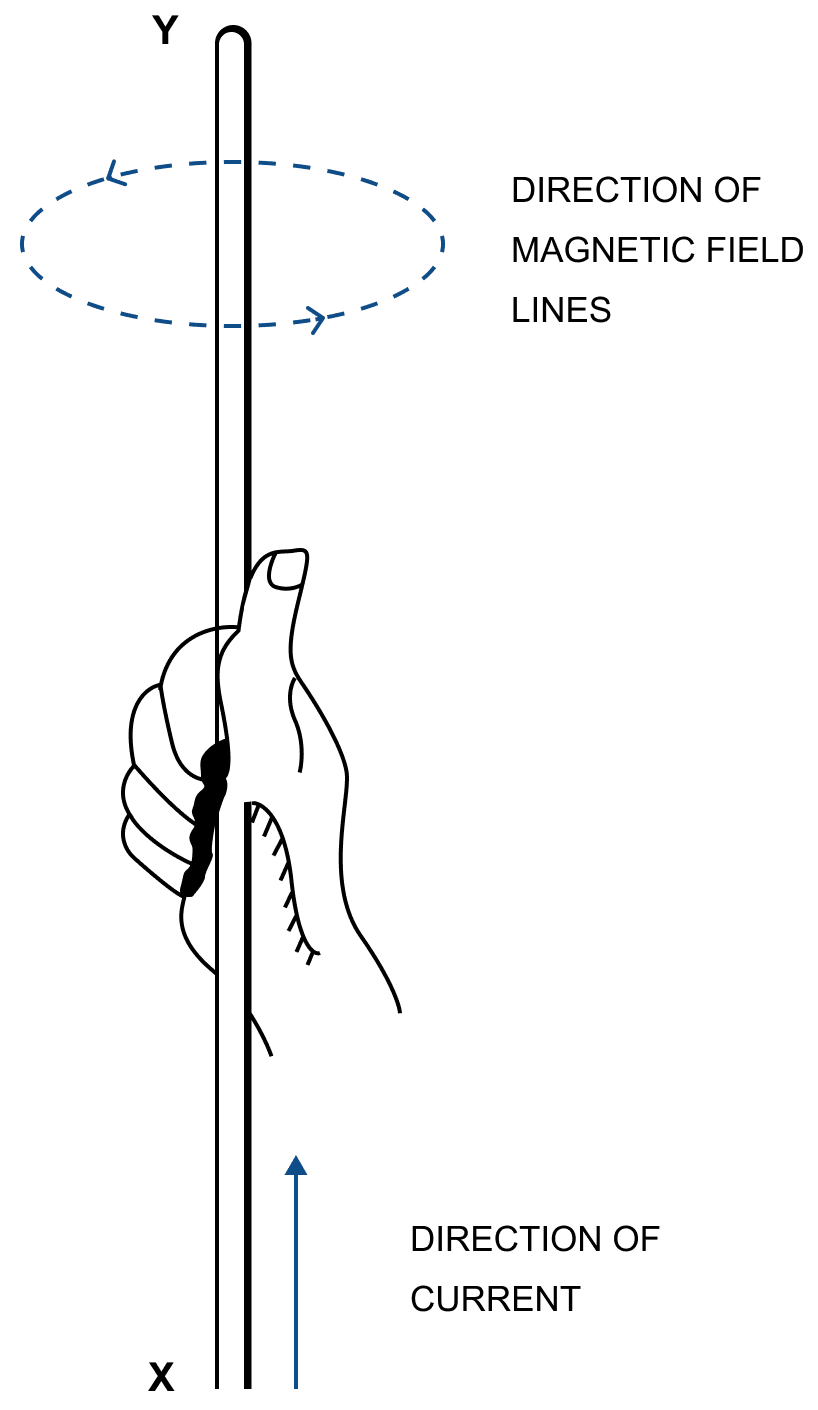In figure, the direction of current in the conductor XY is upwards (i.e., X to Y). The magnetic field lines are anticlockwise around the conductor.

#### Question 5

A straight wire lying in a horizontal plane carries a current from north to south. (a) What will be the direction of magnetic field at a point just underneath it? (b) Name the law used to arrive at the answer in part (a).

(a) When a straight wire is lying in a horizontal plane and carrying current from north to south then the direction of magnetic field at a point just underneath is towards east.

(b) The law used is right hand thumb rule.

#### Question 6

What will happen to a compass needle when the compass is placed below a wire and a current is made to flow through the wire? Give a reason to justify your answer.

When current is made to flow through the wire, a magnetic field is produced around it. When the compass needle is placed below the wire, it experiences a torque due to this magnetic field. So it deflects to align itself in the direction of magnetic field.

#### Question 7

Draw a labelled diagram showing the three magnetic field lines of a loop carrying current. Mark the direction of current and the direction of magnetic field by arrows in your diagram.

Below diagram shows the magnetic field lines of a loop carrying current: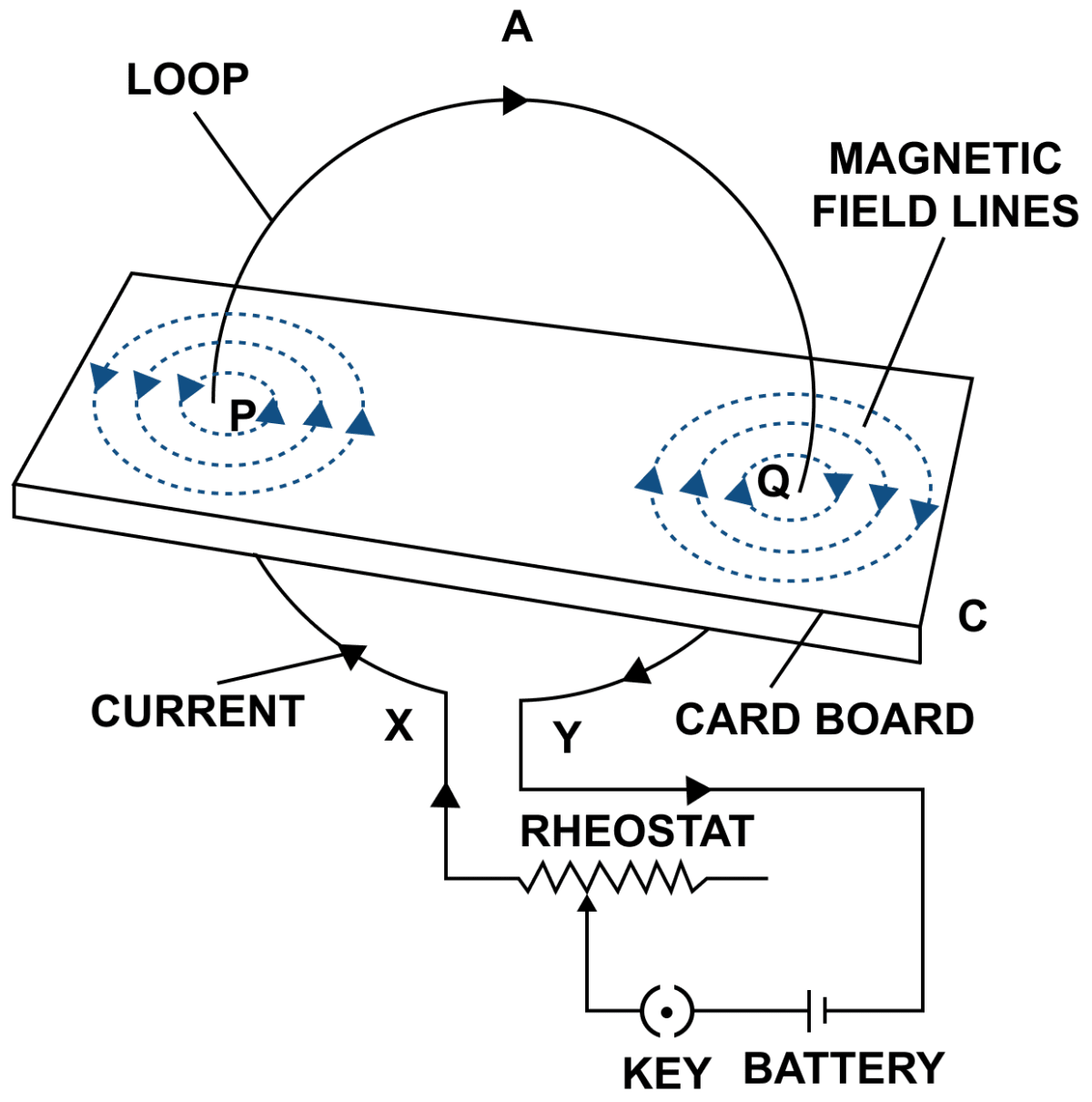#### Question 8

A wire, bent into a circle, carries current in an anticlockwise direction. What polarity does this face of the coil exhibit?

This face of the coil exhibits North polarity.

#### Question 9

What is the direction of magnetic field at the centre of coil carrying current in (i) clockwise, (ii) anticlockwise direction?

(i) The direction of magnetic field at the centre of coil carrying current in clockwise direction is along the axis of coil inwards.

(ii) The direction of magnetic field at the centre of coil carrying current in anticlockwise direction is along the axis of coil outwards.

#### Question 10

Draw a diagram to represent the magnetic field lines along the axis of a current carrying solenoid. Mark arrows to show the direction of current in the solenoid and the direction of magnetic field lines.

Below diagrams shows the magnetic field lines along the axis of a current carrying solenoid with the direction of current and direction of magnetic field lines marked: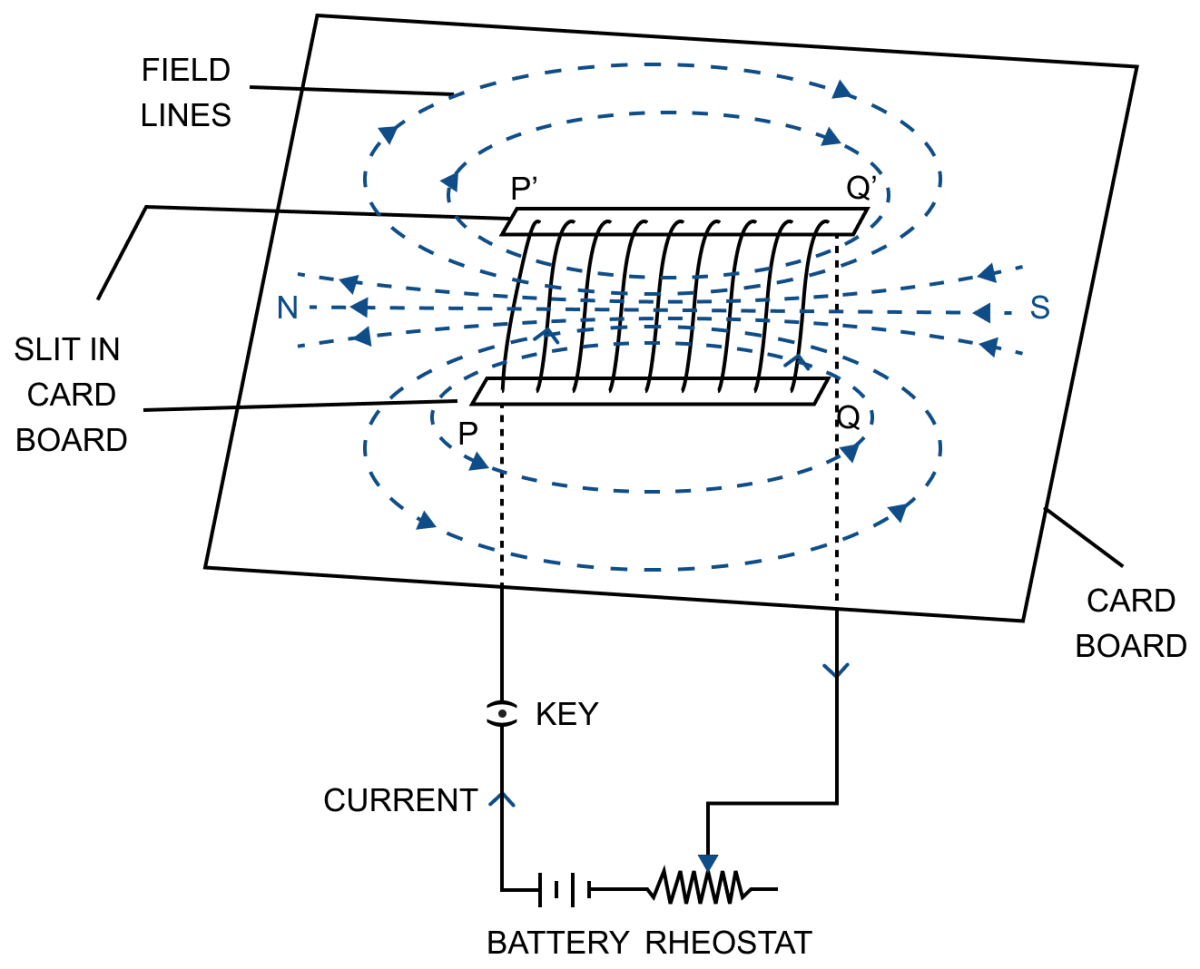#### Question 11

Name and state the rule by which the polarity at the ends of a current carrying solenoid is determined.

Polarity at the ends of a current carrying solenoid is determined by the Clock rule.
It states that looking at the face of the loop, if the current in wire around that face is in anticlockwise direction, the face has the north polarity, while if the current at that face is in clockwise direction, the face has the south polarity.

#### Question 12

The diagram in figure shows a small magnet placed near a solenoid AB with it's north pole N near the end A. Current is switched on in the solenoid by pressing the key K.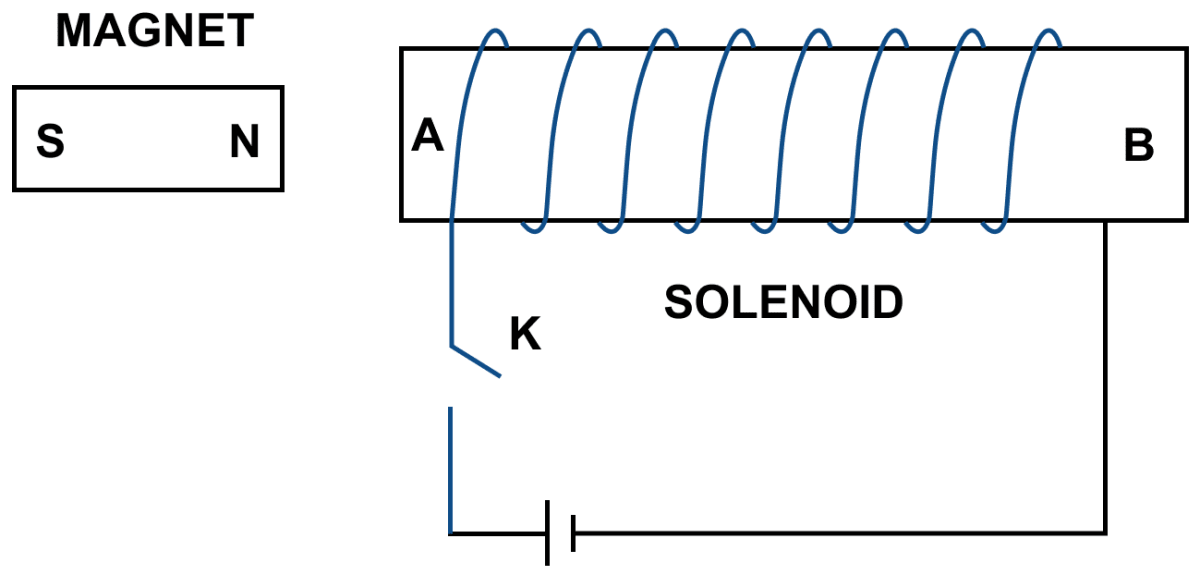(a) State the polarity at the ends A and B.

(b) Will the magnet be attracted or repelled? Give a reason for your answer.

(a) The direction of current in the solenoid at the face A is anticlockwise, so it will have the North polarity and the face B of solenoid at which the current is clockwise will have the South polarity.

(b) The magnet will be repelled because the end A of the solenoid becomes North pole as current at this face is anticlockwise and it repels the north pole of the magnet.

#### Question 13

The diagram in figure shows a spiral coil wound on a hollow cardboard tube AB. A magnetic compass is placed close to it. Current is switched on by closing the key.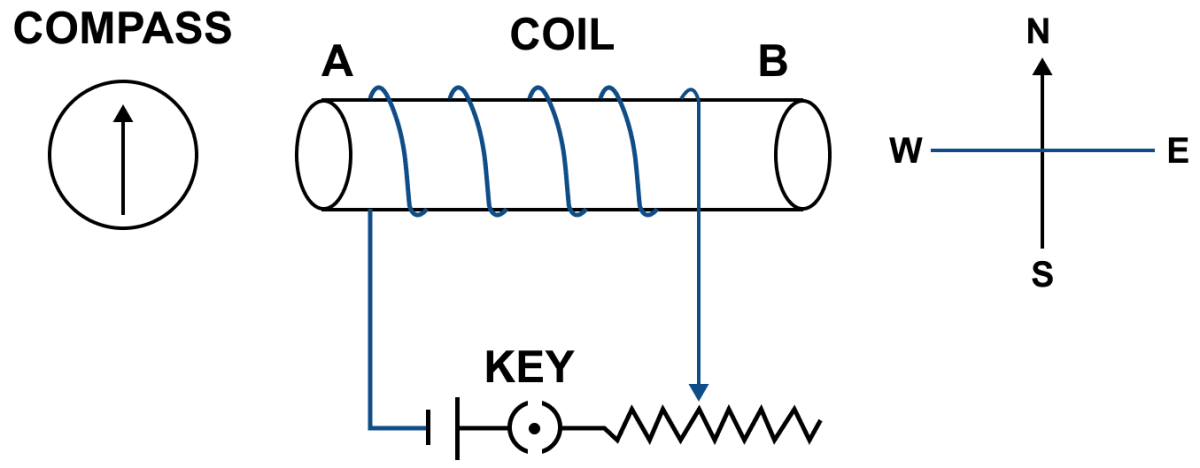(a) What will be the polarity at the ends A and B?

(b) How will the compass needle be affected? Give reason.

(a) With the help of the Right hand thumb rule, we get the polarity at A — North pole and at B — South pole.

(b) The end A of the coil behaves like north pole and repels north pole of compass needle. Hence, the compass needle deflects towards west.

#### Question 14

State two ways by which the magnetic field due to a current carrying solenoid can be made stronger.

The magnetic field due to a current carrying solenoid can be made stronger —

1. By increasing the number of turns of winding in the solenoid
2. By increasing the current through the solenoid.

#### Question 15

Why does a current carrying freely suspended solenoid rest along a particular direction? State the direction in which it rests.

A current carrying solenoid behaves like a bar magnet so it rests itself in geographic North-South direction.

#### Question 16

What effect will there be on a magnetic compass when it is brought near a current carrying solenoid?

The needle of the compass will rest in the direction of magnetic field due to solenoid at that point.

#### Question 17

How is the magnetic field due to a solenoid carrying current affected if a soft iron bar is introduced inside the solenoid?

When a soft iron is introduced inside a solenoid, then the strength of magnetic field increases.

#### Question 18

Complete the following sentences:

(a) When current flows in a wire, it creates ...........

(b) On reserving the direction of current in a wire, the magnetic field produced by it gets ............

(c) A current carrying solenoid behaves like a ............

(d) A current carrying solenoid when freely suspended, it always rest in ........... direction.

(a) When current flows in a wire, it creates a magnetic field around it.

(b) On reserving the direction of current in a wire, the magnetic field produced by it gets reversed.

(c) A current carrying solenoid behaves like a bar magnet.

(d) A current carrying solenoid when freely suspended, it always rest in north-south direction.

#### Question 19

You are required to make an electromagnet from a soft iron bar by using a cell, an insulated coil of copper and a switch. (a) Draw a circuit diagram to represent the process. (b) Label the poles of the electromagnet.

Below circuit diagram shows an electromagnet made from a soft iron bar by using a cell, an insulated coil of copper and a switch with its poles labelled: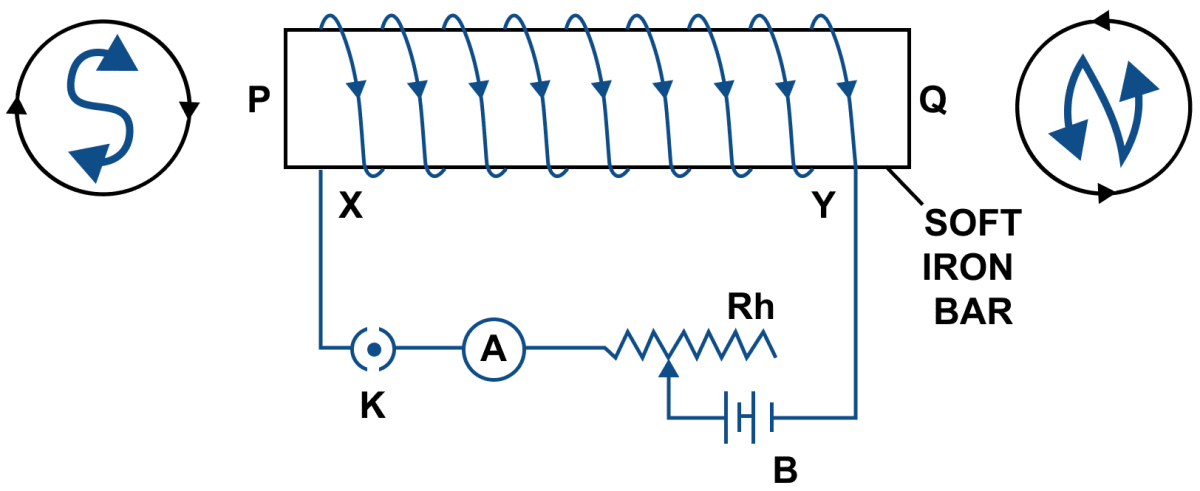#### Question 20

The diagram in figure. shows a coil wound around a soft iron bar XY. (a) State the polarity at the end X and Y as the switch is pressed. (b) Suggest one way of increasing the strength of electromagnet so formed.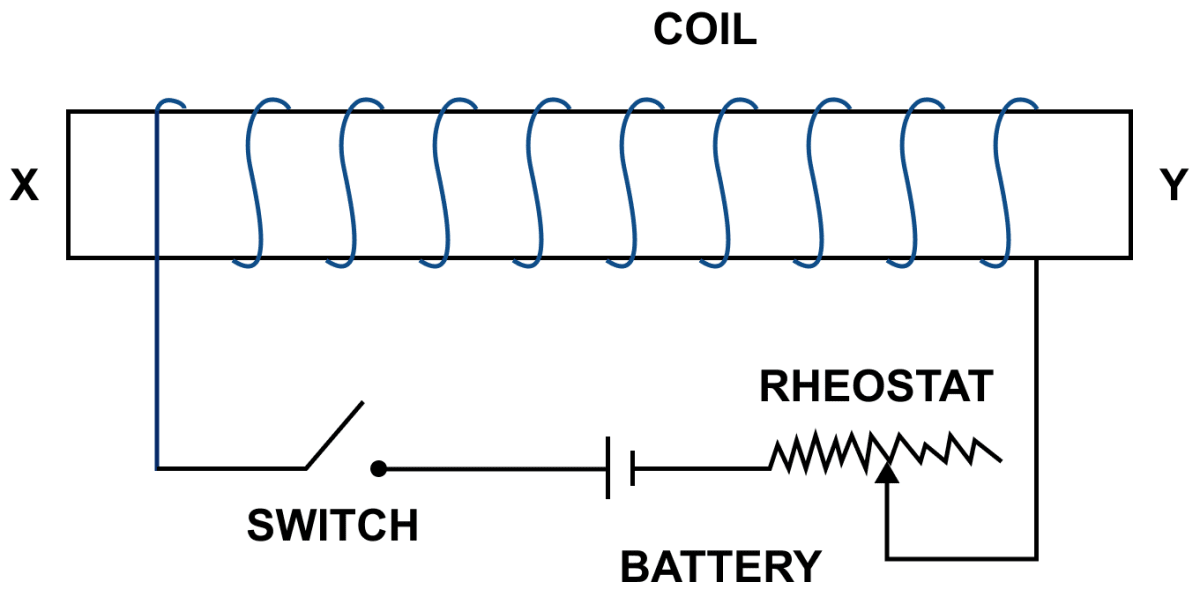(a) When current is passed through the winding of the solenoid, current at end X is in anticlockwise direction so it becomes the North pole. The current at end Y is in clockwise direction so it becomes the South pole.

(b) One way of increasing the strength of electromagnet so formed is by reducing resistance of circuit by means of rheostat to increase current in the coil.

#### Question 21

(a) What name is given to a cylindrical coil of diameter less than it's length?

(b) If a piece of soft iron is placed inside the coil mentioned in part (a) and current is passed in the coil from a battery, what name is then given to the device so obtained?

(c) Give one use of the device mentioned in part (b).

(a) Solenoid is a cylindrical coil of diameter less than its length.

(b) The device so obtained is an electromagnet.

(c) It is used in an electric relay.

#### Question 22

Show with the aid of the diagram how a wire is wound on a U-shaped piece of soft iron in order to make it an electromagnet. Complete the circuit diagram and label the poles of the electromagnet.

Below circuit diagram shows an electromagnet made by winding a wire on a U-shaped piece of soft iron: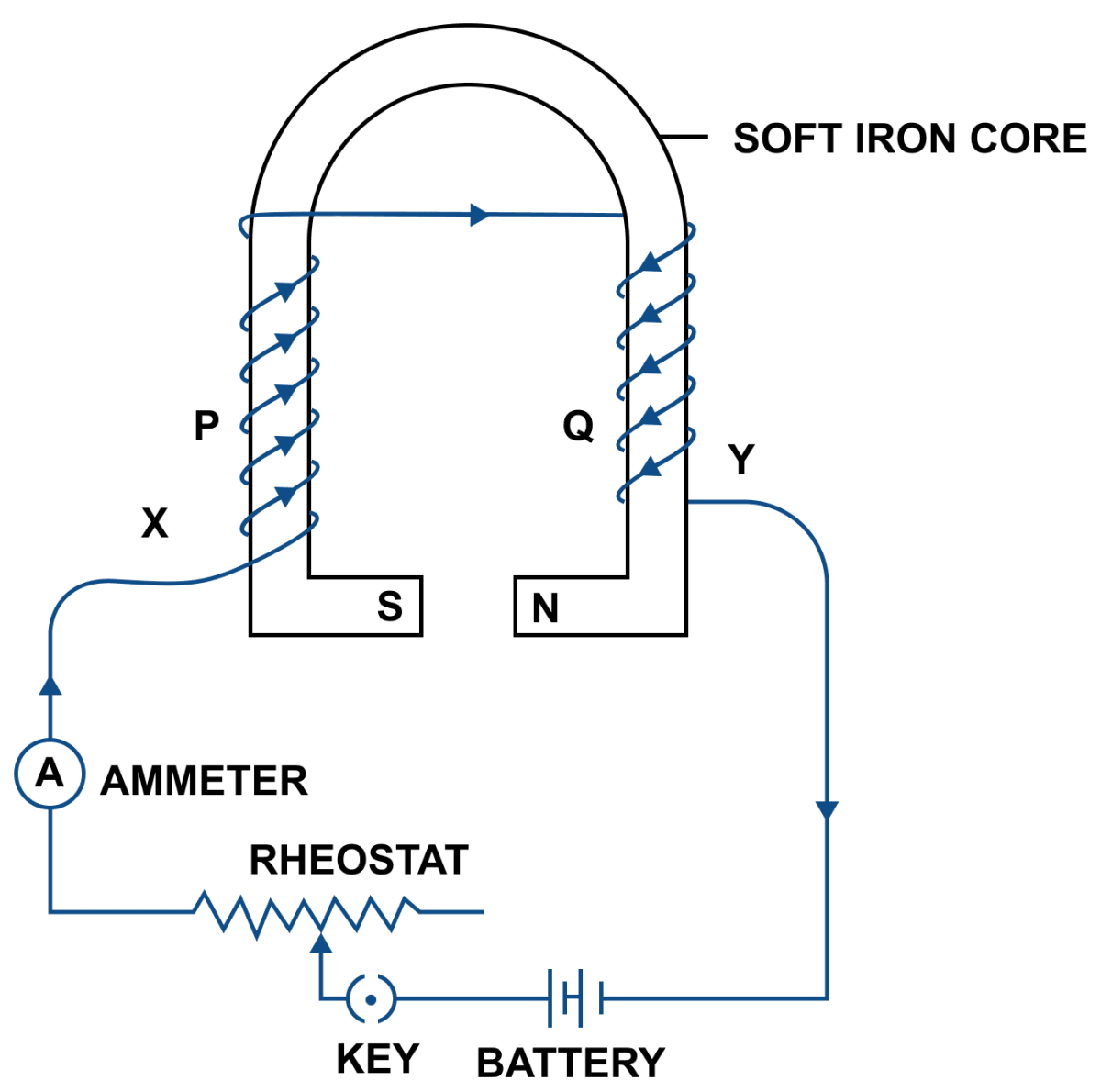#### Question 23

What is an electromagnet? Name two factors on which the strength of magnetic field of an electromagnet depends and state how does it depend on the factors stated by you.

An electromagnet is a temporary strong magnet made by passing current in a coil wound around a piece of soft iron. It is an artificial magnet.

The strength of magnetic field of an electromagnet depends on —

1. Number of turns — By increasing the number of turns of windings in the solenoid, the strength of magnetic field of an electromagnet can be increased.
2. Current — By increasing the current through the solenoid, the strength of magnetic field can be increased.

#### Question 24

Figure shows the current flowing in the coil of wire wound around the soft iron horse shoe core.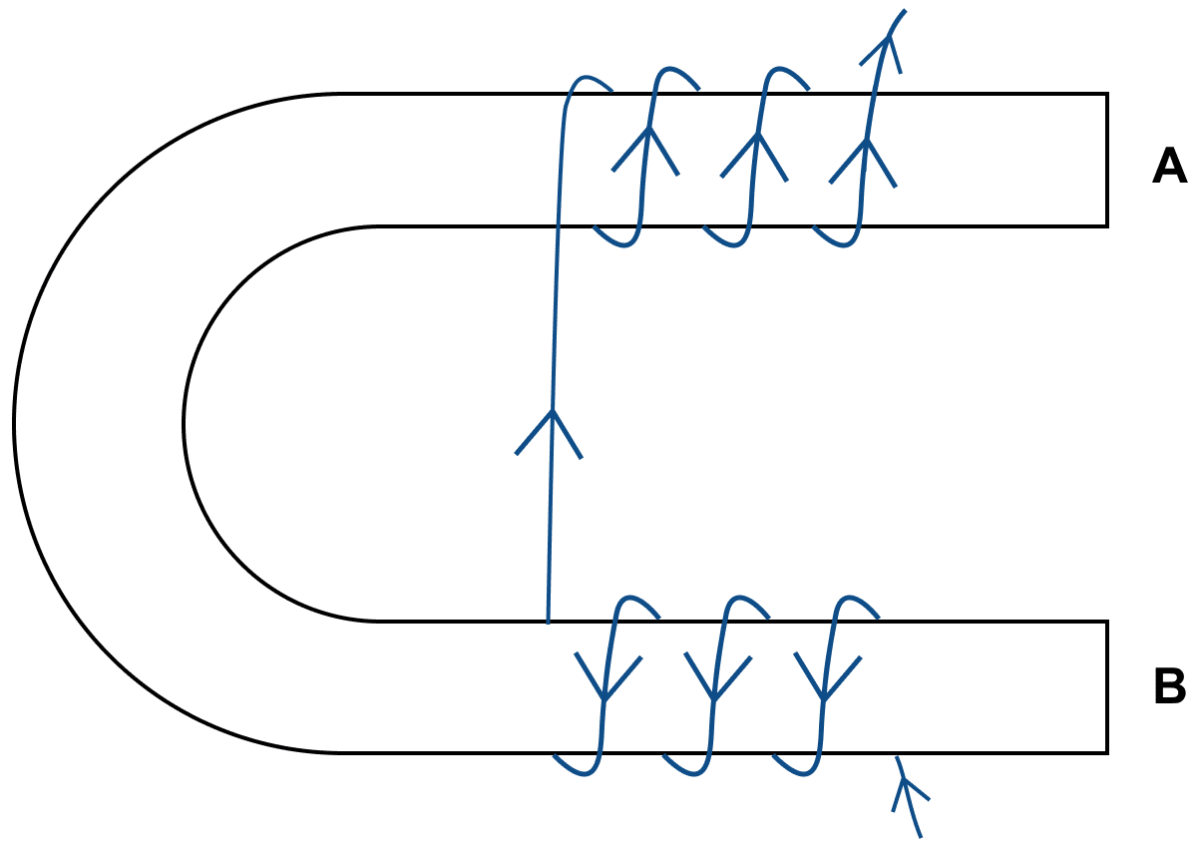(a) State the polarities developed at the ends A and B.

(b) How will the polarity at the ends A and B change on reversing the direction of current.

(c) Suggest one way to increase the strength of magnetic filed produced.

(a) When current is passed through the windings, the end A of the arm becomes south pole (current at this face is clockwise) and the end B becomes north pole (current at this face is in anticlockwise direction).

(b) When the direction of current is reversed then the polarity will also reverse. A will become north pole (current at this face is in anticlockwise direction) and B will become south pole (current at this face is clockwise).

(c) The strength of magnetic field produced can be increased by increasing the current.

#### Question 25

State two ways by which the strength of an electromagnet can be increased.

The strength of an electromagnet can be increased:

1. By increasing the number of turns of winding in the solenoid.
2. By increasing the current through the solenoid.

#### Question 26

Name one device that uses an electromagnet.

An electric bell is one of the common devices that uses an electromagnet.

#### Question 27

State two advantages of an electromagnet over a permanent magnet.

An electromagnet has the following advantages over an electromagnet —

1. An electromagnet can produce a strong magnetic field.
2. The strength of the magnetic field of an electromagnet can easily be changed by changing the current (or the number of turns) in the solenoid.

#### Question 28

State two differences between an electromagnet and a permanent magnet.

Comparison of an electromagnet with a permanent magnet

ElectromagnetPermanent magnet
It is made up of soft iron.It is made up of steel.
It produces the magnetic field as long as current flows in it's coil. i.e., it produces the temporary magnetic field.It produces a permanent magnetic field.

#### Question 29

Why is soft iron used as the core of the electromagnet in an electric bell?

The soft iron bar is used as the core of the electromagnet in an electric bell because it has low retentivity and therefore, the bar remains magnetized so long the electric current flows through the solenoid, but it gets demagnetized as soon as the current is switched off.

#### Question 30

How is the working of an electric bell affected, if alternating current be used instead of direct current?

If an a.c. source is used in place of battery, the core of electromagnet will get magnetized, but the polarity at it's ends will change. Since attraction of armature does not depend on the polarity of electromagnet, so the bell will still ring on pressing the switch.

#### Question 31

The incomplete diagram of an electric bell is given in figure. Draw winding of coil on the core and complete the electric circuit in the diagram.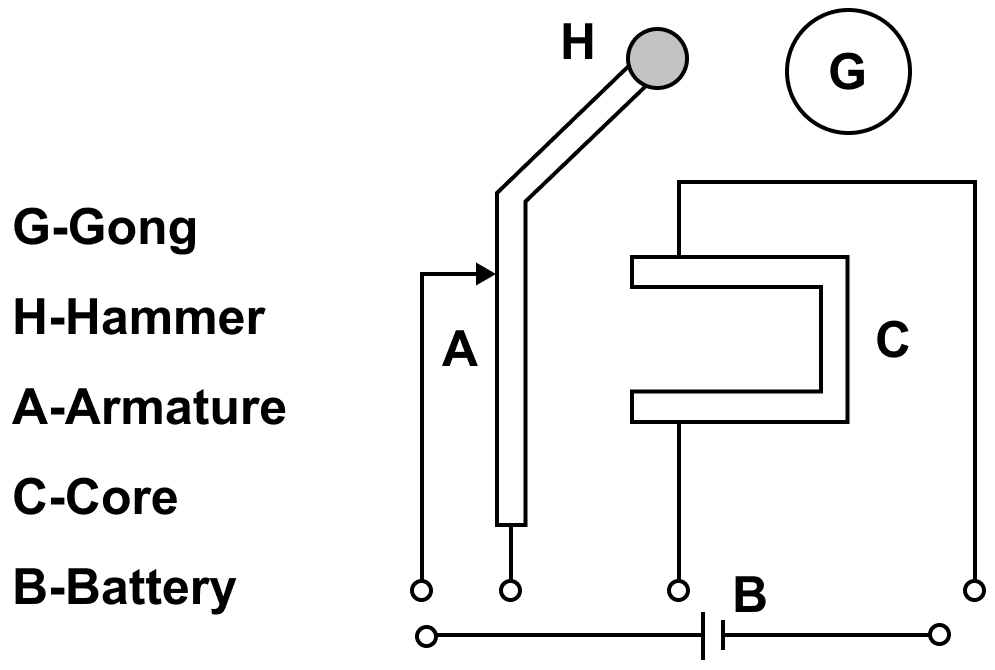The completed diagram of the electric bell with winding of coil drawn is shown below: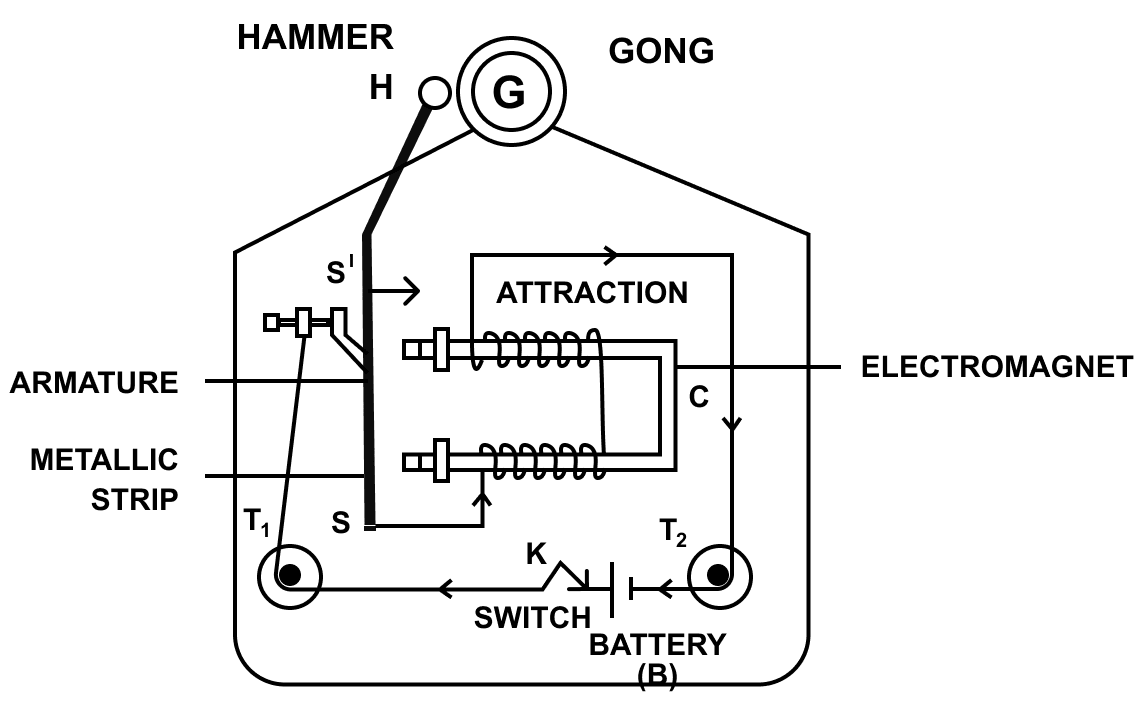#### Question 32

Name the material used for making the armature of an electric bell. Give a reason for your answer.

The material used for making the armature of an electric bell is soft iron.
It is used as the core of the electromagnet in an electric bell because it has low retentivity and therefore, the bar remains magnetized so long as the electric current flows through the solenoid but it gets demagnetized as soon as the current is switched off.

## Multiple Choice Type

#### Question 1

The presence of magnetic field at a point can be detected by means of:

1. a strong magnet
2. a solenoid
3. a compass needle ✓
4. a current carrying wire.

The presence of magnetic field at a point can be detected by means of a compass needle.

In the absence of any magnetic field, the needle of compass can rest in any direction. But in absence of a magnetic field, the needle of compass rests only along the direction of magnetic field. Hence, we can detect the magnetic field with the help of the compass.

#### Question 2

On reversing the direction of current in a wire, the magnetic field produced by it:

1. gets reversed in direction ✓
2. increases in strength
3. decreases in strength
4. remains unchanged in strength and direction.

By reversing the direction of current in a wire, the magnetic field produced by it gets reversed in direction because the polarity of the faces of the wire gets reversed.

## Exercise 10(B)

#### Question 1

Name three factors on which the magnitude of force on a current carrying conductor placed in a magnetic field depends and state how does the force depend on the factors stated by you.

Experimentally, it is found that the magnitude of force acting on a current carrying conductor placed in a magnetic field in the direction perpendicular to it's length, depends on the following three factors —

1. The force F is directly proportional to the strength of magnetic field B, i.e., F α B
2. The force F is directly proportional to the current I flowing in the wire, i.e., F α I
3. The force F is directly proportional to the length L of the wire (within the magnetic field) i.e., F α L

#### Question 2

State condition in each case for the magnitude of force on a current carrying conductor placed in a magnetic field to be (a) zero, (b) maximum

(a) The magnitude of force on a current carrying conductor placed in a magnetic field is zero, when current in conductor is in the direction of magnetic field.

(b) The magnitude of force on a current carrying conductor placed in a magnetic field is maximum, when current in conductor is normal to the magnetic field.

#### Question 3

How will the direction of force be changed, if the current is reversed in the conductor placed in a magnetic field?

When the current is reversed in the conductor placed in a magnetic field, the direction of force is also reversed.

#### Question 4

Name and state the law which is used to determine the direction of force on a current carrying conductor placed in a magnetic field.

The direction of force on a current carrying conductor placed in a magnetic field is obtained by the Fleming's left hand rule.

Fleming's left hand rule — Stretch the forefinger, central finger and the thumb of your left hand mutually perpendicular to each other as shown in figure. If the forefinger indicates the direction of magnetic field and the central finger indicates the direction of current, then the thumb will indicate the direction of motion of conductor.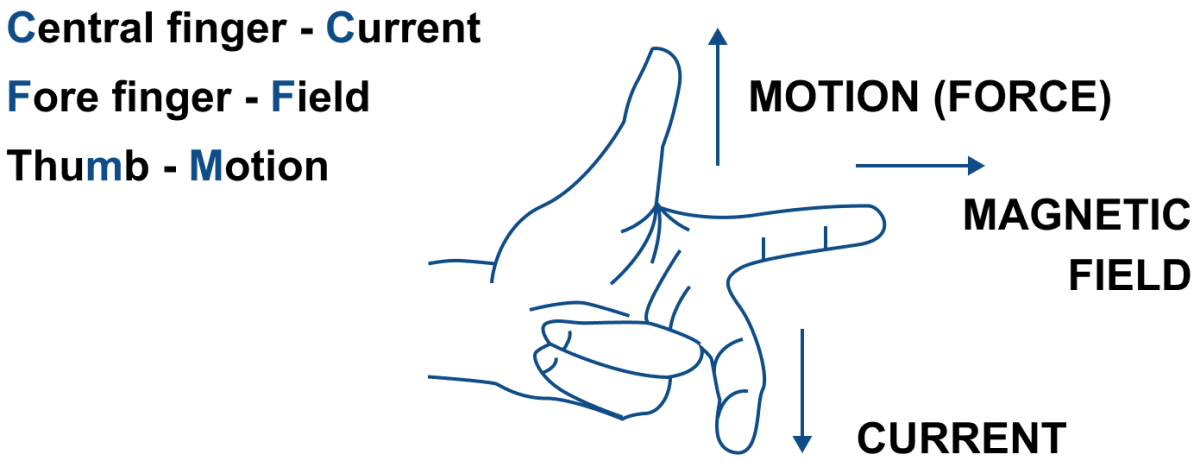#### Question 5

State Fleming's left handle rule.

Fleming's left hand rule — Stretch the forefinger, central finger and the thumb of your left hand mutually perpendicular to each other as shown in figure. If the forefinger indicates the direction of magnetic field and the central finger indicates the direction of current, then the thumb will indicate the direction of motion of conductor.#### Question 6

State the unit of magnetic field in terms of the force experienced by a current carrying conductor placed in a magnetic field.

The unit of magnetic field in terms of the force experienced by a current carrying conductor placed in a magnetic field is —

$\dfrac{\text{Newton}}{\text{ampere x meter}}$ (or N A -1 m-1)

#### Question 7

A flat coil ABCD is freely suspended between the pole pieces of a U-shaped permanent magnet with the plane of coil parallel to the magnetic field.

(a) What happens when a current is passed in the coil?

(b) When will the coil come to rest?

(c) When will the couple acting on the coil be (i) maximum, (ii) minimum?

(d) Name an instrument which makes use of the principle stated above.

(a) When a current is passed in the coil, the coil experiences a torque due to which it rotates.

(b) The coil will come to rest when it's plane becomes normal to the magnetic field.

(c) Maximum — The couple acting on the coil be maximum, when the plane of coil is parallel to the magnetic field.
Minimum — The couple acting on the coil be minimum, when the plane of coil is normal to the magnetic field.

(d) The instrument which makes use of the principle is D.C. Motor.

#### Question 8

A coil ABCD mounted on an axle is placed between the poles N and S of a permanent magnet as shown in figure.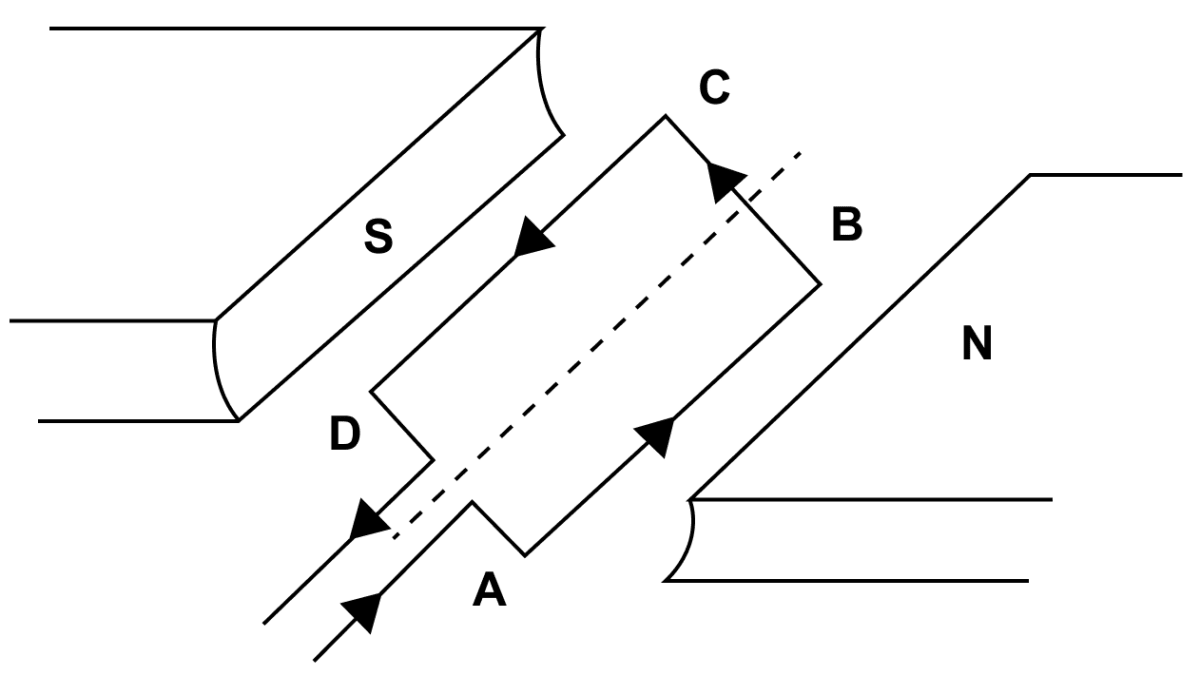(a) In which direction will the coil begin to rotate when current is passed through the coil in direction ABCD by connecting a battery between the ends A and D of the coil?

(b) Why is a commutator necessary for the continuous rotation of coil?

(c) Complete the diagram with commutator, etc. for the flow of current in the coil.

(a) The coil begins to rotate in anticlockwise direction, when current is passed through the coil in direction ABCD by connecting a battery between the ends A and D of the coil.

(b) A commutator is necessary for the continuous rotation of coil because the arms AB and CD get interchanged after half rotation, so the direction of torque on coil reverses. To keep the coil rotating in the same direction, a commutator is needed to reverse the direction of current in the coil after each half rotation of coil.

(c) Completed diagram with commutator showing the flow of current in the coil is given below: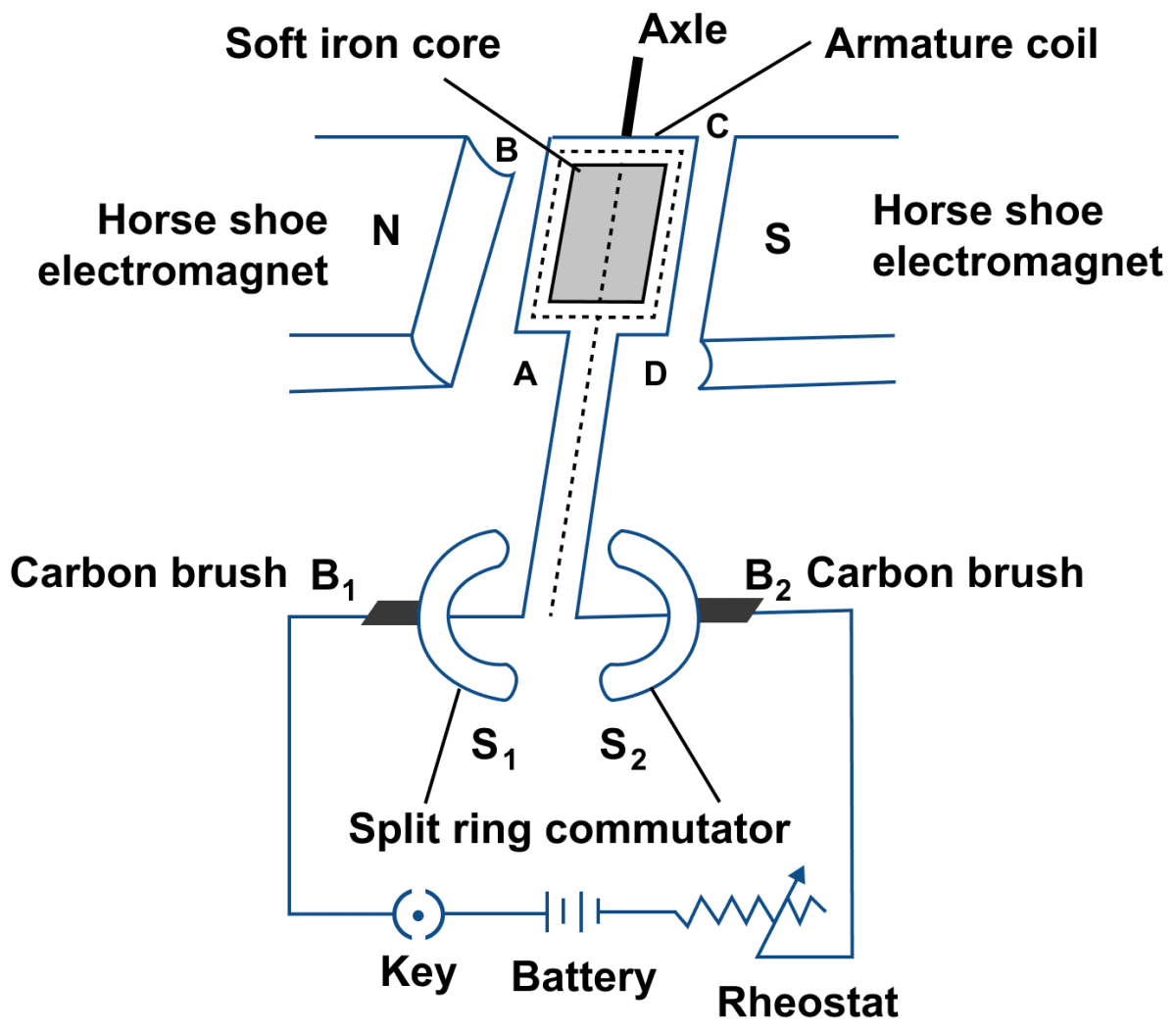#### Question 9

What is an electric motor? State it's principle.

An electric motor is a device which converts the electrical energy into the mechanical energy.

Principle — An electric motor (dc motor) works on the principle that on passing electric current through a conductor placed normally in a magnetic field, a force acts on the conductor as a result of which the conductor begins to move and thus mechanical energy (or work) is obtained.

#### Question 10

Draw a labelled diagram of a d.c motor showing it's main parts.

Below diagram shows a d.c motor with its parts labelled:#### Question 11

What energy conversion does take place during the working of a d.c motor?

During the working of a d.c. motor electrical energy converts into mechanical energy.

#### Question 12

State two ways by which the speed of rotation of an electric motor can be increased.

The speed of rotation of an electric motor can be increased —

1. By increasing the strength of current in the coil.
2. By increasing the number of turns in the coil.

#### Question 13

Name two appliances in which an electric motor is used.

Electric motors are used in electrical gadgets like fan, washing machine, etc.

## Multiple Choice Type

#### Question 1

In an electric motor, the energy transformation is:

1. from electrical to chemical
2. from chemical to light
3. from mechanical to electrical
4. from electrical to mechanical ✓

An electric motor is a device which converts the electrical energy into the mechanical energy.

## Exercise 10(C)

#### Question 1

(a) What is electromagnetic induction?

(b) Describe one experiment to demonstrate the phenomenon of electromagnetic induction.

(a) Whenever there is a change in the number of magnetic field lines linked with a conductor, an electromotive force (e.m.f.) is developed between the ends of the conductor which lasts as long as there is a change in the number of magnetic field lines through the conductor. This phenomenon is called the electromagnetic induction.

(b) Demonstration of the phenomenon of electromagnetic induction —

Experiment — Wind an insulated copper wire in form of a spiral on a paper (or wooden) cylinder so as to form a coil in the form of a solenoid. Connect a centre zero galvanometer G between the two ends of the solenoid. Then, place a magnet NS at some distance along the axis of solenoids as shown in figure.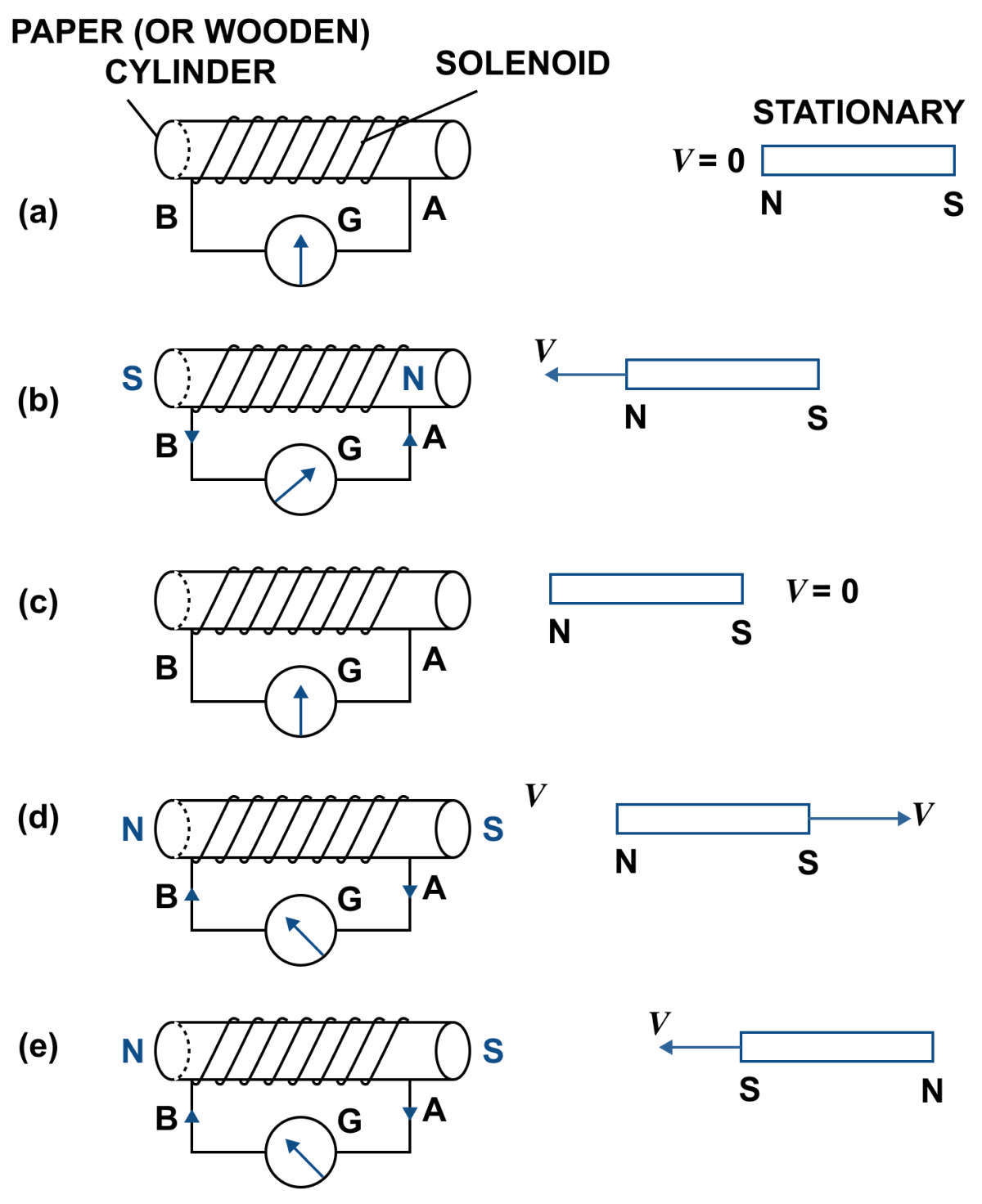Observations — It is observed that,

(i) When the magnet is stationary, there is no deflection in galvanometer and it's pointer reads zero as shown in figure (a).

(ii) When the magnet with it's north pole facing the solenoid is moved towards it, the galvanometer shows a deflection towards right showing that a current flows in solenoid in the direction B to A as shown in figure (b).

(iii) As the motion of magnet is stopped, the pointer of galvanometer comes to zero position. Fig. (c) This shows that the current in solenoid flows as long as the magnet is in motion.

(iv) If the magnet is moved away from the solenoid, the pointer of galvanometer deflects towards left (as shown in figure d) showing that the current in solenoid flows again, but now in direction A to B which is opposite to that as shown in figure b. Current becomes zero as soon as the magnet stops.

(v) If the same action (movement) of magnet is done rapidly, the deflection in the galvanometer is more than before although the direction of deflection remains same. It shows that now more current flows.

(vi) If the magnet is brought towards the solenoid by keeping it's south pole towards it, the pointer of galvanometer deflects towards left as shown in figure (e) showing that the current in solenoid flows in direction A to B which is opposite to that shown in figure (b)

#### Question 2

State Faraday's laws of electromagnetic induction.

Faraday formulated the following two laws of electromagnetic induction —

1. Whenever there is a change in magnetic flux linked with a coil, an e.m.f. is induced. The e.m.f. induced lasts so long there is a change in the magnetic flux linked with the coil.
2. The magnitude of e.m.f. induced is directly proportional to the rate of change of magnetic flux linked with the coil. If magnetic flux changes at a constant rate, a steady e.m.f. is produced.

#### Question 3

State two factor on which the magnitude of induced e.m.f in a coil depend.

The magnitude of induced e.m.f. in a coil depend on following two factors —

1. The rate of change in magnetic flux with each turn of coil, and
2. The number of turns in the coil.

#### Question 4

(a) What kind of the energy change takes place when a magnet is moved towards a coil having a galvanometer between it's ends?

(b) Name the phenomenon.

(a) When a magnet is moved towards a coil having a galvanometer between it's ends then mechanical energy changes to the electrical energy.

(b) The phenomenon is known as Electromagnetic Induction.

#### Question 5

(a) How would you demonstrate that a momentary current can be obtained by the suitable use of a magnet, a coil of wire and a galvanometer?

(b) What is the source of energy associated with the current obtained in part (a)?

(a) When there is a relative motion between the coil and magnet, magnetic flux linked with the coil changes. As shown in figure b, the coil is moved towards the north pole of magnet, so the magnetic flux through the coil increases. Similarly, if the coil is moved away from the north pole of magnet, the magnetic flux through the coil decreases.
Due to change in the magnetic flux linked with the coil, an e.m.f. is induced in the coil. The induced e.m.f. causes a current to flow in the coil if the circuit of coil is closed.

(b) the source of energy associated with the current obtained in part (a) is the Mechanical energy spent in moving the magnet relative to the coil.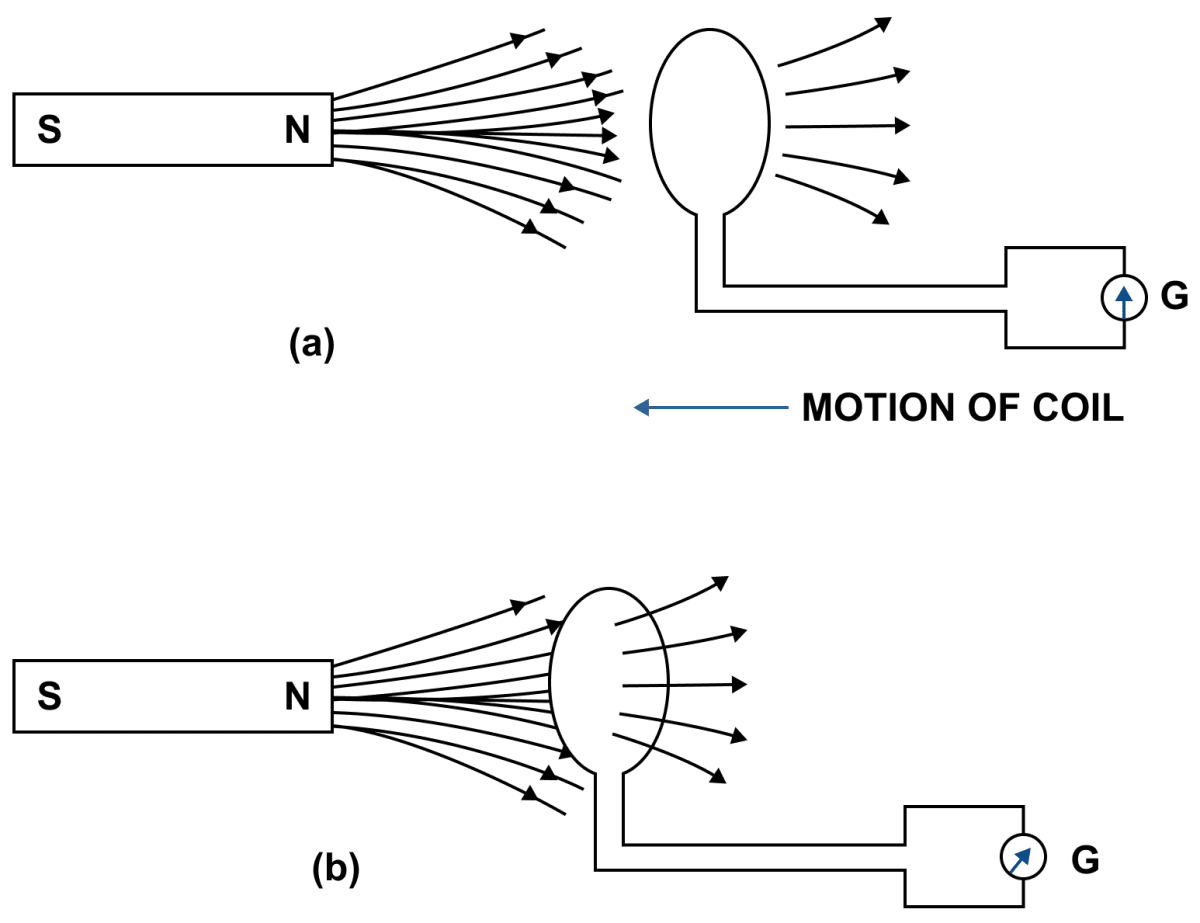#### Question 6

(a) Describe briefly one way of producing an induced e.m.f.

(b) State one factor that determines the magnitude of induced e.m.f. in part (a) above.

(c) What factor determines the direction of induced e.m.f. in part (a) above?

When there is a relative motion between the coil and magnet, magnetic flux linked with the coil changes. As shown in figure b, the coil is moved towards the north pole of magnet, so the magnetic flux through the coil increases. Similarly, if the coil is moved away from the north pole of magnet, the magnetic flux through the coil decreases. Due to change in the magnetic flux linked with the coil, an e.m.f. is induced in the coil. The induced e.m.f. causes a current to flow in the coil if the circuit of coil is closed.(b) The magnitude of induced e.m.f. depends on the rate of change of magnetic flux linked with each turn.

(c) The direction of induced e.m.f. depends on whether there is an increase or decrease in the magnetic flux. It can be determined by any of the following two rules (i) Fleming's right hand rule, (ii) Lenz's law.

#### Question 7

Complete the following sentence —

The current is induced in a closed circuit only if there is _________ .

The current is induced in a closed circuit only if there is change in number of magnetic field lines linked with the circuit.

#### Question 8

In which of the following cases e.m.f. is induced?

(i) A current is started in a wire held near a loop of wire.

(ii) The current is switched off in a wire held near a loop of wire.

(iii) A magnet is moved through a loop of wire.

(iv) A loop of wire is held near a magnet.

(i) Yes, e.m.f. is induced because when current is started in a wire held near a loop of wire, magnetic field varies due to which e.m.f. is induced.

(ii) Yes, e.m.f. is induced because when current is switched off in a wire held near a loop of wire, the current stops and magnetic field again varies and hence e.m.f. is induced.

(iii) Yes, e.m.f. is induced. When there is a relative motion between the magnet and a loop of wire, magnetic flux changes and e.m.f. is induced.

(iv) No, e.m.f. is not induced. When a loop of wire is held near a magnet, there is no change in magnetic field hence e.m.f. is not induced.

#### Question 9

A conductor is moved in a varying magnetic field. Name the law which determines the direction of current induced in the conductor.

When a conductor is moved in a varying magnetic field, then Fleming's right hand rule determines the direction of current induced in the conductor.

Fleming's right hand rule — Stretch the thumb, central finger and forefinger of your right hand mutually perpendicular to each other. If the forefinger indicates the direction of magnetic field and the thumb indicates the direction of motion of the conductor, then the central finger will indicate the direction of induced current.

#### Question 10

State Fleming's right hand rule.

Fleming's right hand rule — Stretch the thumb, central finger and forefinger of your right hand mutually perpendicular to each other. If the forefinger indicates the direction of magnetic field and the thumb indicates the direction of motion of the conductor, then the central finger will indicate the direction of induced current.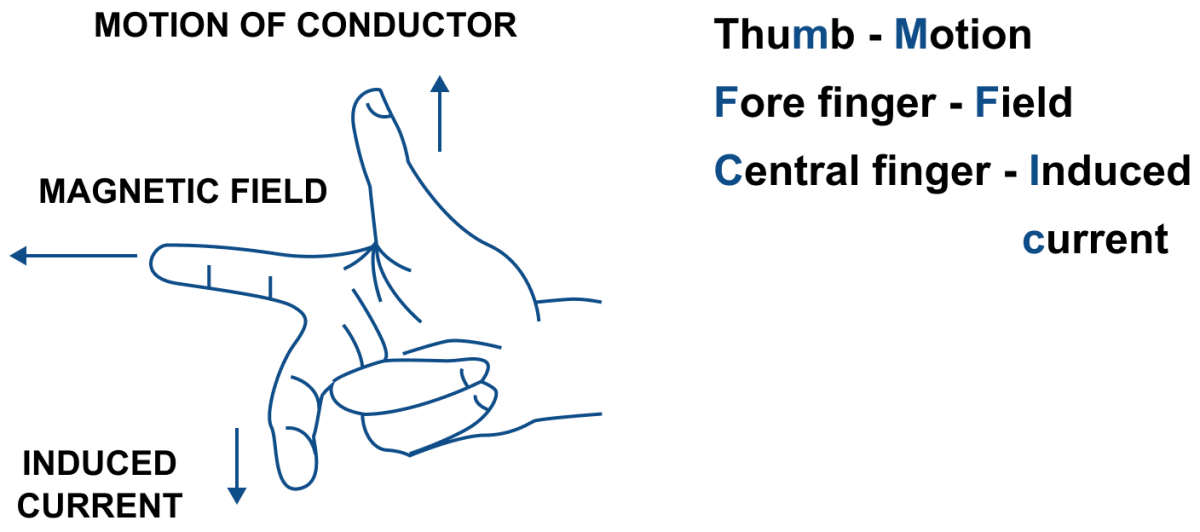#### Question 11

What is Lenz's law?

According to Lenz's law, the direction of induced e.m.f. (or induced current) is such that it opposes the cause which produces it.

#### Question 12

Why does it become more difficult to move a magnet towards a coil when the number of turns in the coil has been increased?

According to Lenz's law, the direction of induced e.m.f. (or induced current) is such that it opposes the cause which produces it. As per Faraday's laws of Electromagnetic Induction, the magnitude of induced e.m.f. is directly proportional to the number of turns in the coil.
Hence, the e.m.f. induced in the coil becomes more when the number of turns in the coil are increased. As a result, a stronger magnetic field is induced that opposes the motion of magnet towards the coil with a greater force making it more difficult to move the magnet towards the coil.

#### Question 13

Explain why an induced current must flow in such a direction so as to oppose the change producing it.

Due to law of conservation of energy, the mechanical energy spent in producing the change is transformed into the electrical energy in form of the induced current. Hence, the induced current flows in a direction to oppose the change so that some mechanical energy is constantly required to overcome the opposing force which in turn will keep the induced current flowing.

#### Question 14

Explain how does the Lenz's law show the conservation of energy in the phenomenon of electromagnetic induction.

Lenz's law is based on the law of conservation of energy. It shows that the mechanical energy is spent in doing work against the opposing force experienced by the moving magnet, and it is transformed into the electrical energy due to which current flows in the solenoid.

#### Question 15

The diagram shows a coil of several turns of copper wire near a magnet NS. The coil is moved in the direction of arrow shown in the diagram.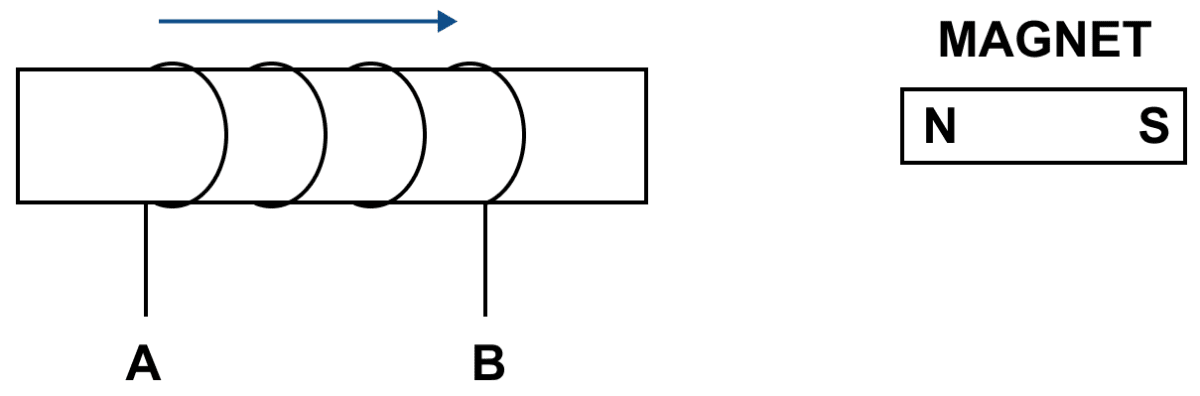(i) In what direction does the induced current flow in the coil?

(ii) Name the law used to arrive at the conclusion in part (i).

(iii) How would the current in coil be altered if:

1. the coil has twice the number of turns,
2. the coil was made to move three times fast?

(i) When north pole of the magnet is brought towards the end B of the solenoid, the induced current flows in the solenoid in direction A to B i.e., at the end B, the current is anticlockwise, so the end B of the solenoid becomes a north pole to repel the magnet. Thus it opposes the motion of north pole of the magnet towards the solenoid, which is the cause producing it.

(ii) The law used to arrive at the conclusion in part (i) is Lenz's law.

(iii) (a) Current is directly proportional to the number of turns. Hence, current becomes twice when the coil has twice the number of turns.

(b) The current in coil becomes thrice when the coil was made to move three times fast .

#### Question 16

The diagram, shows a fixed coil of several turns connected to a centre zero galvanometer G and a magnet NS which can move in the direction shown in the diagram.

(a) Describe the observation in the galvanometer if (i) the magnet is moved rapidly, (ii) the magnet is kept stationary after it has moved into the coil, (iii) the magnet is then rapidly pulled out of the coil.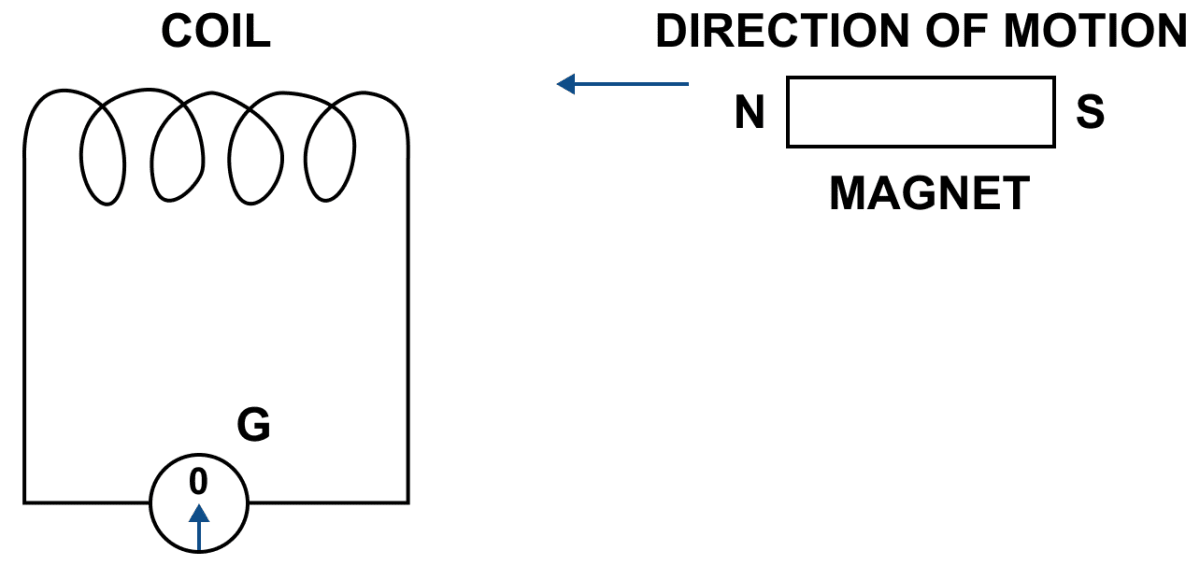(b) How would the observation in (i) of part (a) change if a more powerful magnet is used?

(a) (i) A deflection is observed in the galvanometer towards the right when the magnet is moved away rapidly.

(ii) The deflection becomes zero, when the magnet is kept stationary after it has moved into the coil.

(iii) The deflection again occurs in opposite direction, when the magnet is rapidly pulled out of the coil.

(b) When a more powerful magnet is used then the deflection is increased.

#### Question 17

Name and state the principle of a simple a.c. generator. What is it's use?

An A.C. generator is a device which converts the mechanical energy into the electrical energy using the principle of electromagnetic induction.

Principle — In a generator, a coil is rotated in a magnetic field. Due to rotation, the magnetic flux linked with the coil changes and therefore an e.m.f. is induced between the ends of the coil. Thus, a generator acts as a source of current in an external circuit containing load when connected between the ends of it's coil.

Use — We use a.c. generator in our house as an alternative source of electricity when electric supply from mains is not available.

#### Question 18

What determines the frequency of a.c. produced in a generator?

The frequency of rotations of the coil (i.e., the number of rotations of the coil per second) determines the frequency of a.c. produced in a generator.

#### Question 19

Complete the sentence —

An a.c. generator changes the ___________ energy to ___________ energy.

An a.c. generator changes the mechanical energy to electrical energy.

#### Question 20

Draw a labelled diagram of a simple a.c generator.

Labelled diagram of a simple a.c generator is given below: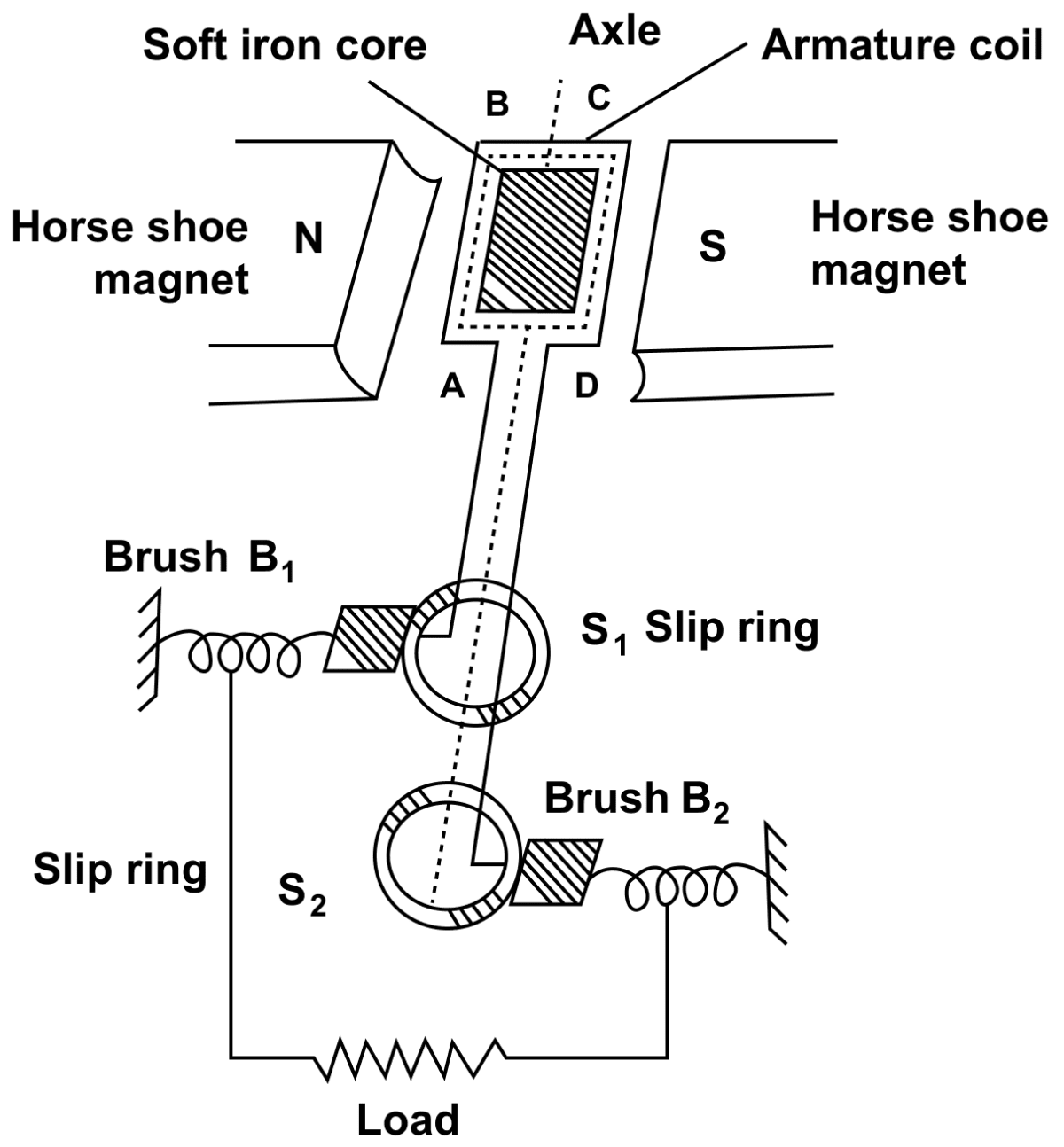#### Question 21

In an a.c generator the speed at which the coil rotates is doubled. How would this affect (a) the frequency of output voltage (b) the maximum output voltage.

(a) When in an a.c generator the speed at which the coil rotates is doubled the frequency of output voltage is also doubled.

(b) The maximum output voltage is also doubled when the speed at which the coil rotates is doubled.

#### Question 22

State two ways to produce a higher e.m.f. in an a.c generator.

Two ways to produce a higher e.m.f. in an a.c. generator are —

1. By increasing the speed of rotation of coil.
2. By increasing the number of turns in the coil.

#### Question 23

What energy conversion does take place in a generator when it is in use?

The mechanical energy changes into the electrical energy in a generator when it is in use.

#### Question 24

State (i) two dis-similarities, and (ii) two similarities between a d.c. motor and an a.c. generator.

(i) Dis-similarities between a d.c. motor and an a.c. generator are —

D.C. motorA.C. generator
A d.c. motor is a device which converts the electrical energy into the mechanical energy.A generator is a device which converts the mechanical energy into the electrical energy.
A d.c. motor works on the principle of force acting on a current carrying conductor placed in a magnetic field.A generator works on the principle of electromagnetic induction.

(ii) Similarities between a d.c. motor and an a.c. generator are —

(i) Both in d.c. motor and an a.c. generator, a coil rotates in a magnetic field between the pole pieces of a powerful electromagnet.

(ii) Both in a d.c. motor and an a.c. generator, there is a transformation of energy from one form to the other form.

#### Question 25

State one advantage of using an a.c. over the d.c.

The advantage of a.c. over d.c. is that it is cheaper and easy to generate a.c. than d.c.

#### Question 26

For what purpose are the transformers used? On which type of current do transformers work?

Transformers are used to step up or step down the a.c. voltage. They work on a.c. current.

#### Question 27

State two factors on which the magnitude of an induced e.m.f. in the secondary coil of a transformer depends.

Factors on which the magnitude of an induced e.m.f. in the secondary coil of a transformer depends are —

1. The turns ratio i.e., the number of turns in the secondary coil to the number of turns in the primary coil.
2. The input voltage.

#### Question 28

How are the e.m.f. in the primary and secondary coils of a transformer related with the number of turns in these coils?

E.m.f. in the primary and secondary coils of a transformer are related with the number of turns in these coils as follows —

$\dfrac{E_s}{E_p}$ = $\dfrac{N_s}{N_p}$

where s stands for secondary, p stands for primary, E is E.m.f and N is number of turns in the coils .

#### Question 29

Draw a labelled diagram to show the various components of a step up transformer.

Below diagrams shows the various components of a step up transformer: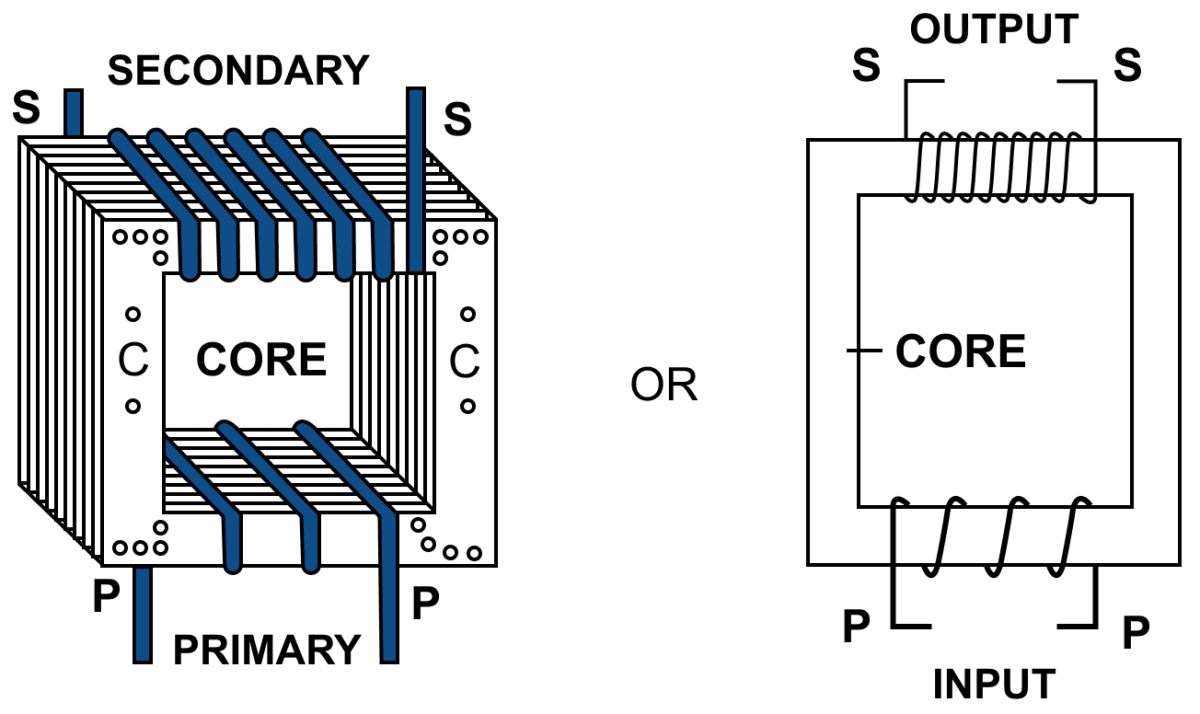#### Question 30

Name the device used to transform 12 V a.c. to 200 V a.c. Name the principle on which it works.

The device used to transform 12 V a.c. to 200 V a.c. is step up transformer. It works on the principle of electromagnetic induction.

#### Question 31

Draw a labelled diagram of a step up transformer and explain how does it work. State two characteristics of the primary coil as compared to it's secondary coil.

Labelled diagram of a step up transformer is given below:The transformer used to change a low alternating voltage to a high alternating voltage (of same frequency) is called a step up transformer (i.e. Es > Ep).

When the terminals of primary coil are connected to the source of alternating e.m.f. a varying current flows through the primary coil. This varying current produces a varying magnetic field in the core of the transformer.Thus the magnetic flux linked with the secondary coil vary due to which an e.m.f. is induced in it. The induced e.m.f. varies in the same manner as the applied e.m.f. in the primary coil varies and thus the induced e.m.f. has the same frequency as that of the applied e.m.f.

In a step up transformer the number of turns in the secondary coil is more than the number of turns in the primary coil ( Ns > Np) i.e., turns ratio n > 1 as shown above.

Since current in primary coil is more than in the secondary coil (or Ip > Is), so the wire in the primary coil is kept thicker than in the secondary coil.

Two characteristics of the primary coil as compared to it's secondary coil are —

1. The number of turns in the primary coil are less than that of the secondary coil.
2. The wire of the primary coil is thicker than that of the secondary coil.

#### Question 32

Draw a labelled diagram of a device you would use to transform 220 V a.c. to 15 V a.c. Name the device and explain how does it work. Give it's two uses.

The labelled diagram of a device used to transform 220 V a.c. to 15 V a.c. is given below: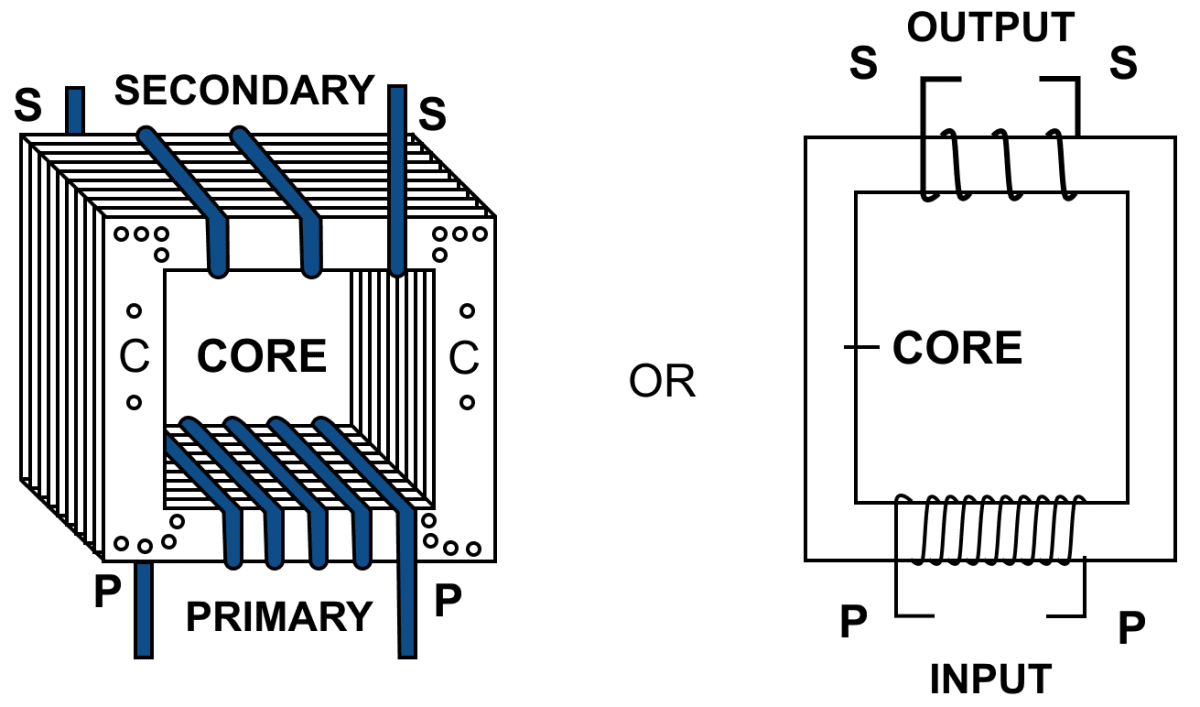Name of this device is Step Down Transformer. It is used to change a high alternating voltage to a low alternating voltage (of same frequency).

When the terminals of primary coil are connected to the source of alternating e.m.f. a varying current flows through the primary coil. This varying current produces a varying magnetic field in the core of the transformer. Thus the magnetic flux linked with the secondary coil vary due to which an e.m.f. is induced in it. The induced e.m.f. varies in the same manner as the applied e.m.f. in the primary coil varies and thus the induced e.m.f. has the same frequency as that of the applied e.m.f.

In a step down transformer the number of turns in the secondary coil are less than the number of turns in the primary coil (Ns < Np) i.e., turns ratio n < 1 as shown in figure. Since current in secondary coil is more than in the primary coil (i.e., Is > Ip ) so the wire in secondary coil is kept thicker than in the primary coil.

Uses of a step down transformer —

1. With electric bells, night electric bulbs, mobile phones, computers etc.
2. At the power sub-stations to step down the voltage before it's distribution to the consumers.

#### Question 33

Name the coil of which the wire is thicker in a (i) step up, (ii) step down transformer. Give reason to your answer.

(i) Step up transformer — The wire in the primary coil is thicker than that in the secondary coil. It is done because the current in the primary coil is more than in the secondary coil and a thick wire results in less resistance and therefore less loss of energy as heat.

(ii) Step down transformer — The wire in the secondary coil is thicker than that in the primary coil. It is done so because the current in the secondary coil is more than in the primary coil and a thick wire results in less resistance and therefore less loss of energy as heat.

#### Question 34

(a) Complete the following diagram in figure of a transformer and name the parts labelled A and B.

(b) Name the part you have drawn to complete the diagram in part (a)

(c) What is the material of the part named above ?

(d) Is this transformer a step-up or stepdown ? Give reason.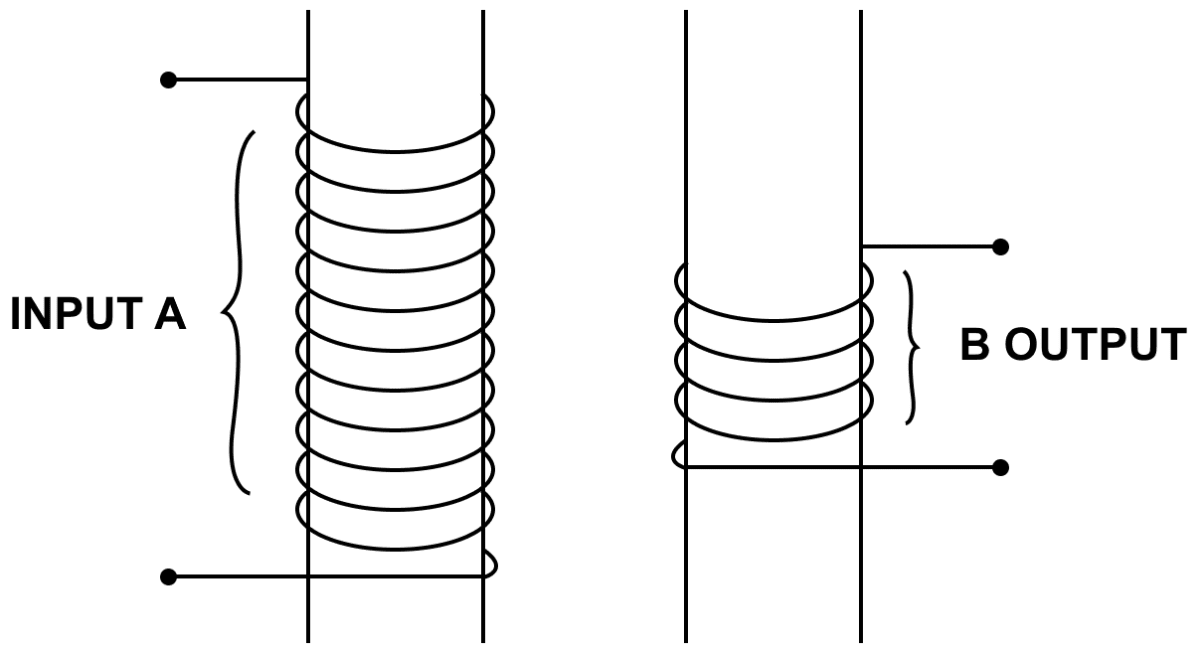(a) Completed and labelled diagram is shown below: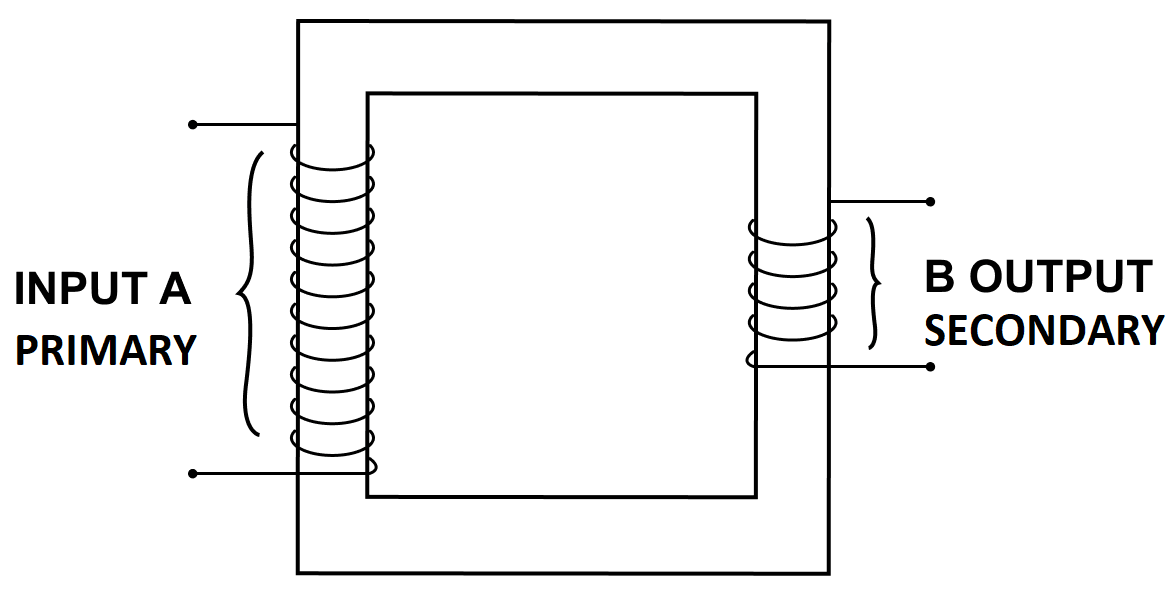(b) The part drawn is the core.

(c) It is a soft iron core.

(d) This is a step down transformer because the number of turns in the secondary coil are less than the primary coil.

#### Question 35

The diagram in figure shows the core of a transformer and it's input and output connections.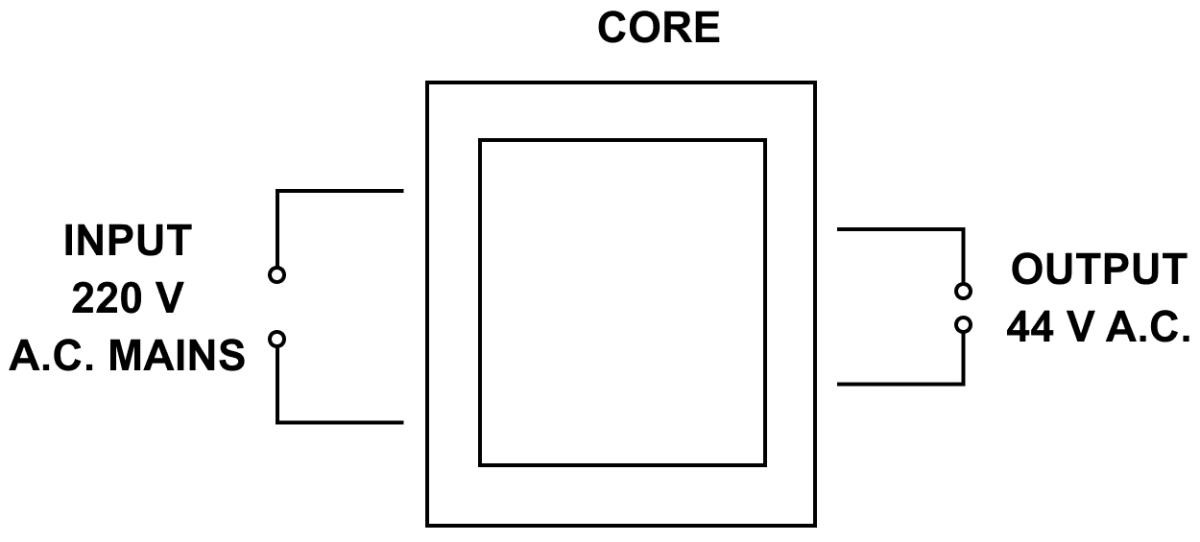(a) State the material used for the core and describe it's structure.

(b) Complete the diagram of the transformer and connections by labelling all parts joined by you.

(c) Name the transformer : step up or step down.

(a) The material used for the core is soft iron. It is made up from thin laminated sheets of soft iron of T and U shape, placed alternately one above the other and insulated from each other by paint (or varnish) coating over them.

(b) Completed diagram is shown below: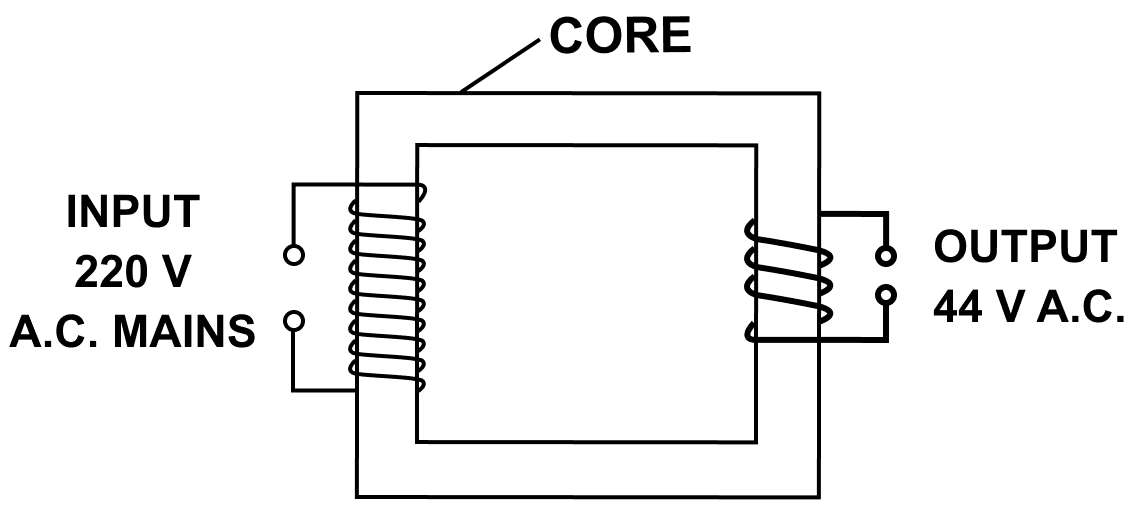(c) As 220 V is converted to 44 V hence, it is a step down transformer.

#### Question 36

The output current of a transformer in which the voltage is stepped down is usually higher than the input current. Explain why.

For an ideal transformer, input power is equal to the output power. From relation P = VI, (where P is power, V is voltage and I is current) , we know that V and I are inversely related. In a step down transformer voltage is decreased and in order to keep the power same the output current increases.

#### Question 37

Why is the iron core of a transformer made laminated (thin sheets) instead of being in one solid piece.

The laminated core prevents the loss of energy due to induced (or eddy) currents in the core. Hence, it is made laminated (thin sheets) instead of being in one solid piece.

#### Question 38

Complete the following sentences —

(i) In a step up transformer, the number of turns in the primary are ..... than the number of turns in the secondary.

(ii) The transformer is used in .............. current circuits.

(iii) In a transformer, the frequency of a.c. voltage ......... (increase / decreases / remains same)

(i) In a step up transformer, the number of turns in the primary are less than the number of turns in the secondary.

(ii) The transformer is used in alternating current circuits.

(iii) In a transformer, the frequency of a.c. voltage remains same. (increase/ decrease/ remains same)

#### Question 39

How do the input and output powers in a transformer compare. State the assumption made.

For an ideal transformer, when there is no energy loss, the output power will be equal to the input power. i.e., power in secondary coil = power in primary coil or EsIs = EpIp

Assumption made is that the resistance is negligible and there is no energy loss.

#### Question 40

Name two kinds of energy losses in a transformer. How are they minimized?

The two kinds of energy losses are —

1. Loss of energy due to induced (or eddy) currents. The use of laminated core prevents this loss of energy.
2. Hysteresis loss — The use of soft iron for the core helps in minimization of this loss.

#### Question 41

Give two points of difference between a step up and step down transformer.

Difference between a step up transformer and step down transformer are:

Step up transformerStep down transformer
It increases the a.c. voltage and decreases the current. i.e., Es > Ep and Is < Ip.It decreases the a.c. voltage and increases the current i.e., Es < Ep and Is > Ip.
The wire in the primary coil is thicker than that of the secondary coil.The wire of the secondary coil is thicker than the primary coil.

#### Question 42

Name the material of the core in (a) an electric bell, (b) electromagnet, (c) a d.c. motor, (d) an a.c. generator, and (e) a transformer.

The material used in —

(a) an electric bell — soft iron core

(b) electromagnet — soft iron core

(c) a d.c. motor — soft iron core

(d) an a.c. generator — soft iron core

(e) a transformer — soft iron core

#### Question 43

Name the transformer used in the (i) power generating station, (ii) power sub station. State the function of each transformer.

The transformer used in the —

(i) Power generating station — Step up transformer is used in Power generating station to increase the alternating voltage from 11 kV to 132 kV.

(ii) Power sub station — Step down transformer is used in Power sub station to decrease the alternating voltage from 132 kV to 33 kV and then further to 11 kV and to 220 V.

## Multiple Choice Type

#### Question 1

The direction of induced current is obtained by:

1. Fleming's left hand rule
2. Clock rule
3. Right hand thumb rule
4. Fleming's right hand rule ✓

The direction of induced current is obtained by Fleming's right hand rule.

Fleming's right hand rule — Stretch the thumb, central finger and forefinger of your right hand mutually perpendicular to each other. If the forefinger indicates the direction of magnetic field and the thumb indicates the direction of motion of the conductor, then the central finger will indicate the direction of induced current.

#### Question 2

In a step up transformer:

1. Ns = Np
2. Ns < Np
3. Ns > Np
4. nothing can be said

In a step up transformer the number of turns in the secondary coil is more than the number of turns in the primary coil ( Ns > Np) i.e., turns ratio n > 1. It increases the a.c. voltage and decreases the current. i.e., Es > Ep and Is < Ip.

## Numericals

#### Question 1

The magnetic flux through a coil having 100 turns decreases from 5 milli weber to zero in 5 second. Calculate the e.m.f. induced in the coil.

Given,

Number of turns (N) = 100 turns

Decrease in the flux = 5 mili weber (0.005 weber) to 0,

Time = 5 second

From relation,

e.m.f. induced e = N × rate of increase of magnetic flux

Substituting the values in the formula we get,

$e = 100 \times \dfrac{0.005}{5} \\[0.5em] \Rightarrow e = 0.1 V \\[0.5em]$

Hence, the e.m.f. induced in the coil = 0.1 V = 100 m V

#### Question 2

The primary coil of a transformer has 800 turns and the secondary coil has 8 turns. It is connected to a 220 V a.c. supply. What will be the output voltage?

Given,

Np = 800

Ns = 8

Ep = 220 V

Es = ?

Now,

$\dfrac{E_s}{E_p} = \dfrac{N_s}{N_p}$

Substituting the values in the formula we get,

$\dfrac{E_s}{220} = \dfrac{8}{800} \\[0.5em] \Rightarrow E_s = 220 \times \dfrac{8}{800} \\[0.5em] \Rightarrow E_s = 2.2 V \\[0.5em]$

Hence, output voltage = 2.2 V

#### Question 3

A transformer is designed to give a supply of 8 V to ring a house bell from the 240 V a.c mains. The primary coil has 4800 turns. How many turns will be in the secondary coil?

Given,

Es = 8 V

Ep = 240 V

Np = 4800

Ns = ?

We know that,

Now,

$\dfrac{E_s}{E_p} = \dfrac{N_s}{N_p}$

Substituting the values in the formula we get,

$\dfrac{8}{240} = \dfrac{N_s}{4800} \\[0.5em] \Rightarrow N_s = 4800 \times \dfrac{8}{240} \\[0.5em] \Rightarrow N_s = 160 \\[0.5em]$

Hence, the number of turns in the secondary coil = 160

#### Question 4

The input and output voltages of a transformer are 220 V and 44 V respectively. Find (a) the turns ratio (b) the current in input circuit if the output current is 2 A.

(a) Given,

Ep = 220 V

ES = 44 V

Now,

$\dfrac{E_s}{E_p} = \dfrac{N_s}{N_p}$

Substituting the values in the formula we get,

$\dfrac{44}{220} = \dfrac{N_s}{N_p} \\[0.5em] \Rightarrow \dfrac{N_s}{N_p} = \dfrac{44}{220} \\[0.5em] \Rightarrow \dfrac{N_s}{N_p} = \dfrac{1}{5} \\[0.5em]$

Hence, turns ratio = 1 : 5

(b) Is = 2 A

Ip = ?

From relation,

${E_s}{I_s} = {E_p}{I_p}$

Substituting the values in the formula we get,

$44 \times 2 = 220 \times I_p \\[0.5em] \Rightarrow I_p = \dfrac{44 \times 2}{220} \\[0.5em] \Rightarrow I_p = 0.4 A \\[0.5em]$

Hence, input current = 0.4 A